Скачать презентацию Numerical Algorithms x x-1 0 1 1 2

fd80ecb7f1caf9a4e33b76a5f9e55b71.ppt

• Количество слайдов: 89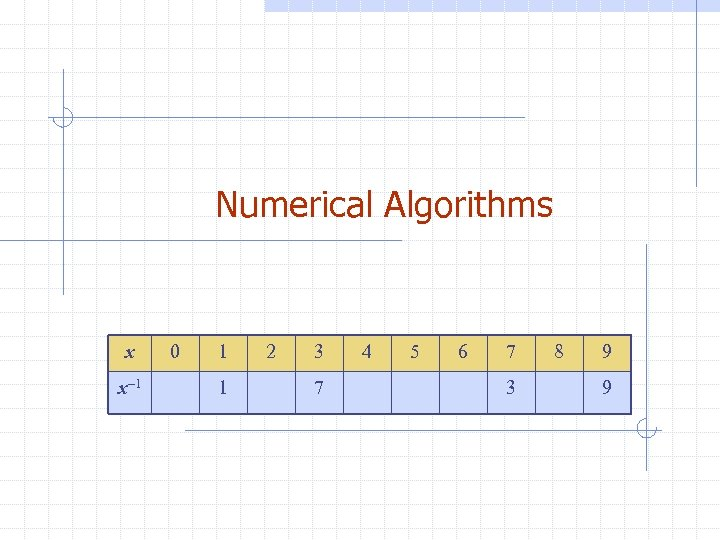Numerical Algorithms x x-1 0 1 1 2 3 7 4 5 6 7 3 8 9 9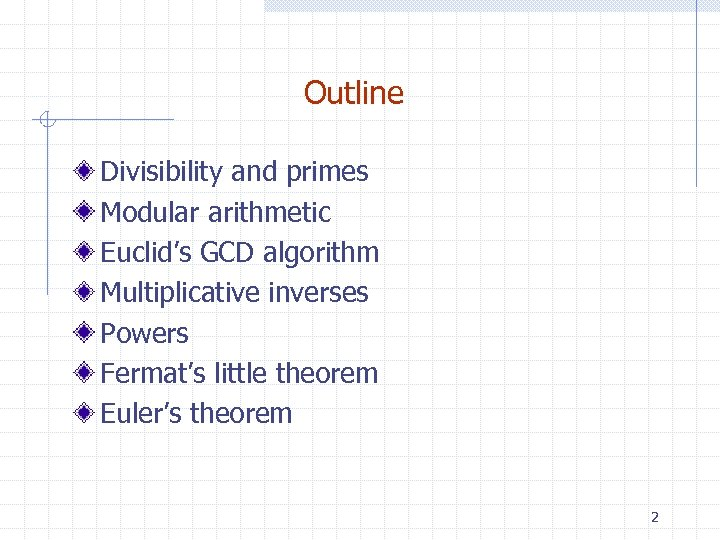Outline Divisibility and primes Modular arithmetic Euclid’s GCD algorithm Multiplicative inverses Powers Fermat’s little theorem Euler’s theorem 2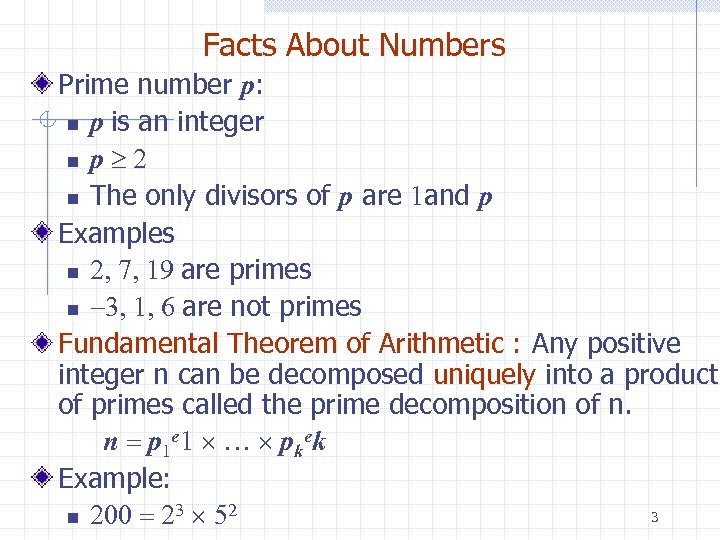Facts About Numbers Prime number p: n p is an integer n p 2 n The only divisors of p are 1 and p Examples n 2, 7, 19 are primes n -3, 1, 6 are not primes Fundamental Theorem of Arithmetic : Any positive integer n can be decomposed uniquely into a product of primes called the prime decomposition of n. n = p 1 e 1 … pkek Example: 3 n 200 = 23 52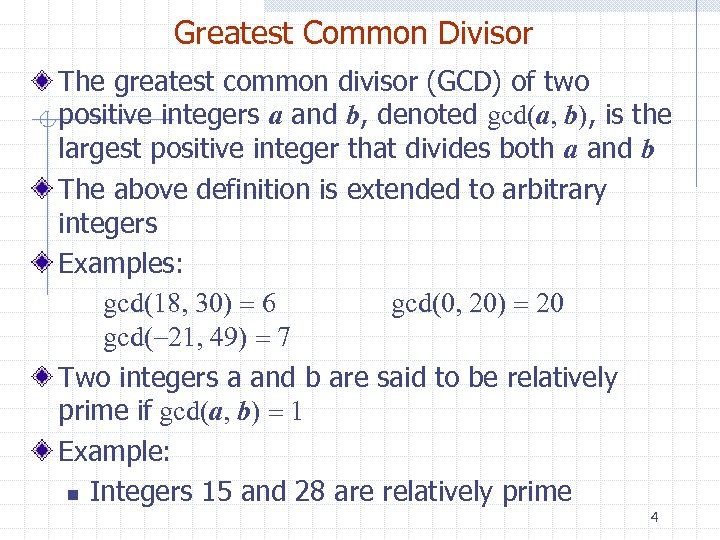Greatest Common Divisor The greatest common divisor (GCD) of two positive integers a and b, denoted gcd(a, b), is the largest positive integer that divides both a and b The above definition is extended to arbitrary integers Examples: gcd(18, 30) = 6 gcd(0, 20) = 20 gcd(-21, 49) = 7 Two integers a and b are said to be relatively prime if gcd(a, b) = 1 Example: n Integers 15 and 28 are relatively prime 4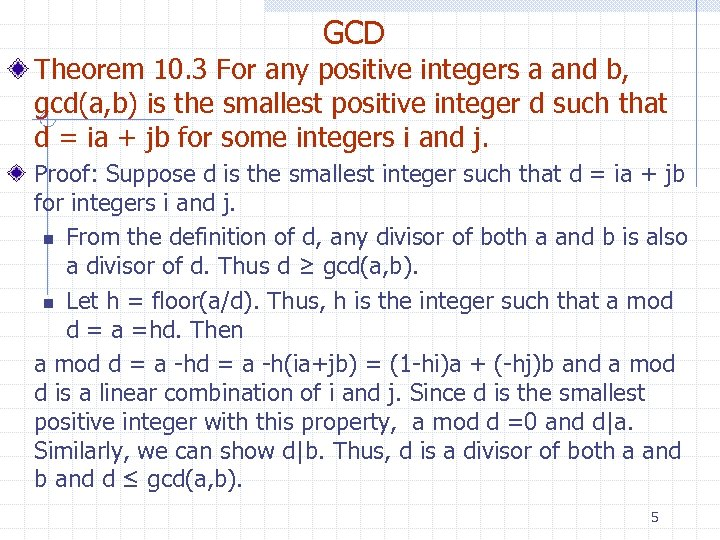GCD Theorem 10. 3 For any positive integers a and b, gcd(a, b) is the smallest positive integer d such that d = ia + jb for some integers i and j. Proof: Suppose d is the smallest integer such that d = ia + jb for integers i and j. n From the definition of d, any divisor of both a and b is also a divisor of d. Thus d ≥ gcd(a, b). n Let h = floor(a/d). Thus, h is the integer such that a mod d = a =hd. Then a mod d = a -h(ia+jb) = (1 -hi)a + (-hj)b and a mod d is a linear combination of i and j. Since d is the smallest positive integer with this property, a mod d =0 and d|a. Similarly, we can show d|b. Thus, d is a divisor of both a and b and d ≤ gcd(a, b). 5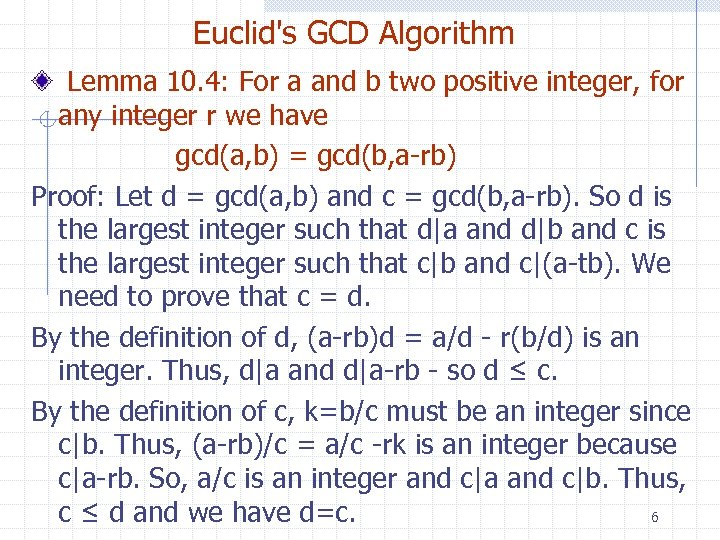Euclid's GCD Algorithm Lemma 10. 4: For a and b two positive integer, for any integer r we have gcd(a, b) = gcd(b, a-rb) Proof: Let d = gcd(a, b) and c = gcd(b, a-rb). So d is the largest integer such that d|a and d|b and c is the largest integer such that c|b and c|(a-tb). We need to prove that c = d. By the definition of d, (a-rb)d = a/d - r(b/d) is an integer. Thus, d|a and d|a-rb - so d ≤ c. By the definition of c, k=b/c must be an integer since c|b. Thus, (a-rb)/c = a/c -rk is an integer because c|a-rb. So, a/c is an integer and c|a and c|b. Thus, 6 c ≤ d and we have d=c.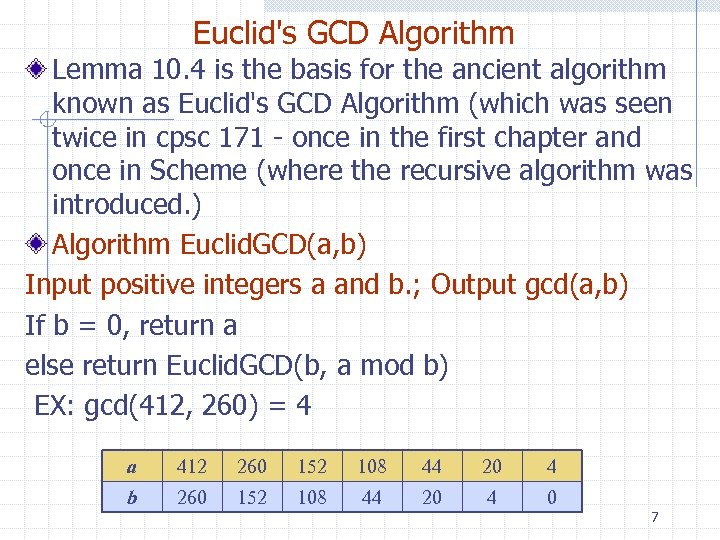Euclid's GCD Algorithm Lemma 10. 4 is the basis for the ancient algorithm known as Euclid's GCD Algorithm (which was seen twice in cpsc 171 - once in the first chapter and once in Scheme (where the recursive algorithm was introduced. ) Algorithm Euclid. GCD(a, b) Input positive integers a and b. ; Output gcd(a, b) If b = 0, return a else return Euclid. GCD(b, a mod b) EX: gcd(412, 260) = 4 a 412 260 152 108 44 20 4 b 260 152 108 44 20 4 0 7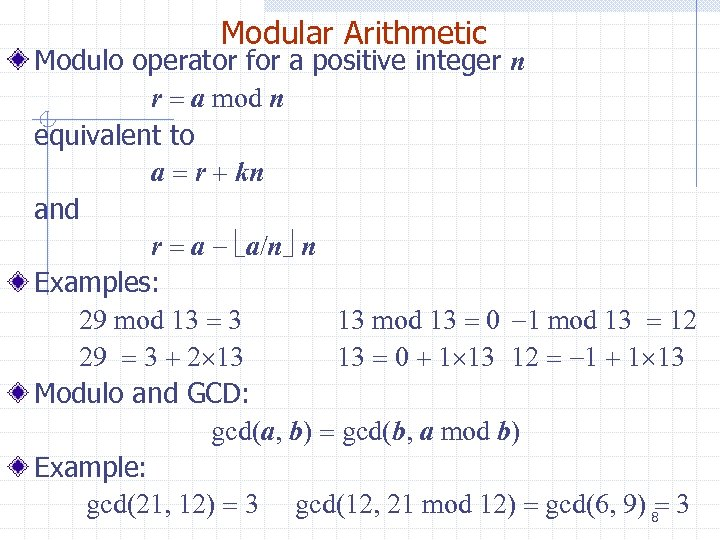Modular Arithmetic Modulo operator for a positive integer n r = a mod n equivalent to a = r + kn and r = a - a/n n Examples: 29 mod 13 = 3 13 mod 13 = 0 -1 mod 13 = 12 29 = 3 + 2 13 13 = 0 + 1 13 12 = -1 + 1 13 Modulo and GCD: gcd(a, b) = gcd(b, a mod b) Example: gcd(21, 12) = 3 gcd(12, 21 mod 12) = gcd(6, 9) 8= 3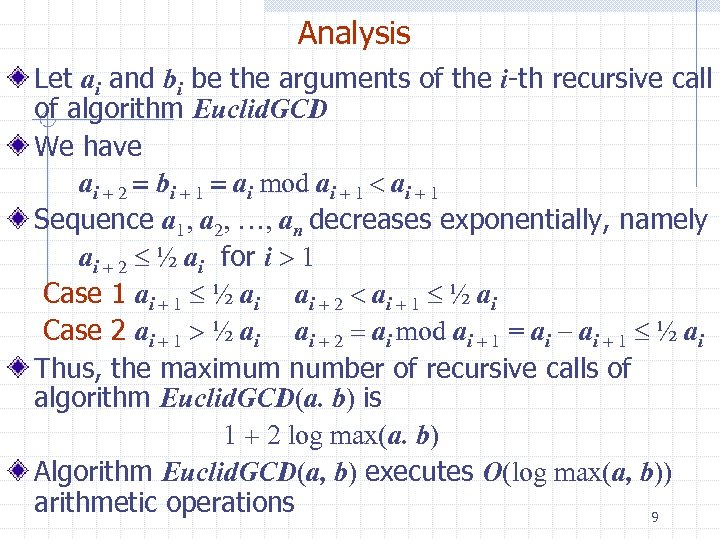Analysis Let ai and bi be the arguments of the i-th recursive call of algorithm Euclid. GCD We have ai + 2 = bi + 1 = ai mod ai + 1 < ai + 1 Sequence a 1, a 2, …, an decreases exponentially, namely ai + 2 ½ ai for i > 1 Case 1 ai + 1 ½ ai ai + 2 < ai + 1 ½ ai Case 2 ai + 1 > ½ ai ai + 2 = ai mod ai + 1 = ai - ai + 1 ½ ai Thus, the maximum number of recursive calls of algorithm Euclid. GCD(a. b) is 1 + 2 log max(a. b) Algorithm Euclid. GCD(a, b) executes O(log max(a, b)) arithmetic operations 9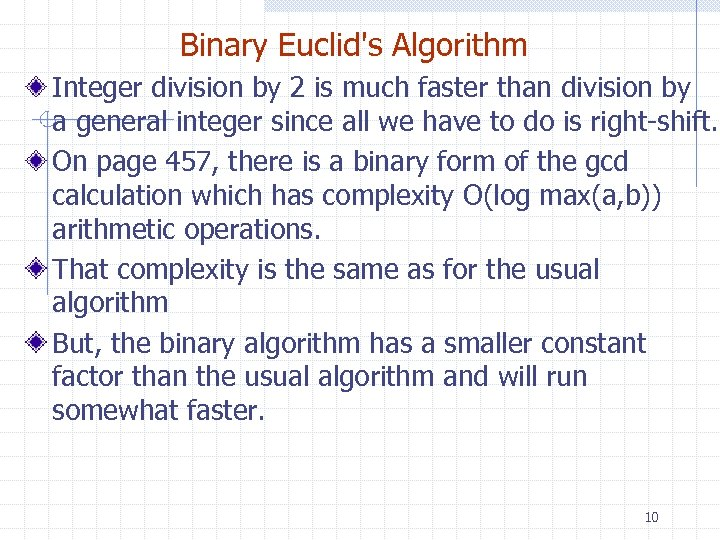Binary Euclid's Algorithm Integer division by 2 is much faster than division by a general integer since all we have to do is right-shift. On page 457, there is a binary form of the gcd calculation which has complexity O(log max(a, b)) arithmetic operations. That complexity is the same as for the usual algorithm But, the binary algorithm has a smaller constant factor than the usual algorithm and will run somewhat faster. 10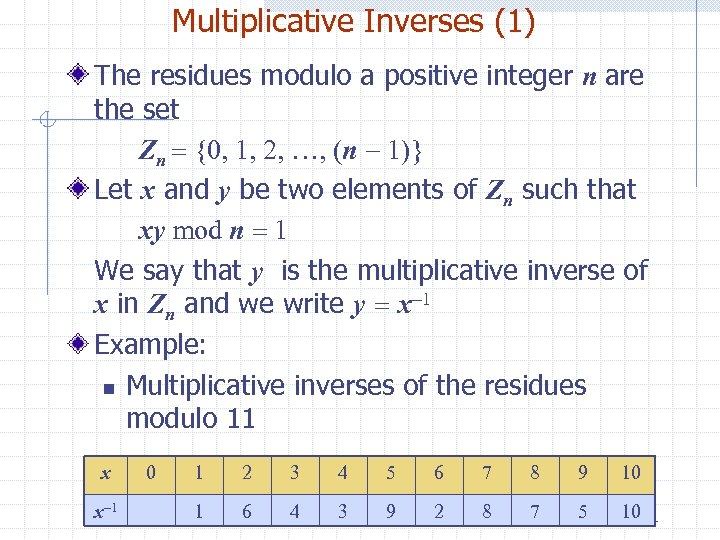Multiplicative Inverses (1) The residues modulo a positive integer n are the set Zn = {0, 1, 2, …, (n - 1)} Let x and y be two elements of Zn such that xy mod n = 1 We say that y is the multiplicative inverse of x in Zn and we write y = x-1 Example: n Multiplicative inverses of the residues modulo 11 x x-1 0 1 2 3 4 5 6 7 8 9 10 1 6 4 3 9 2 8 7 5 10 11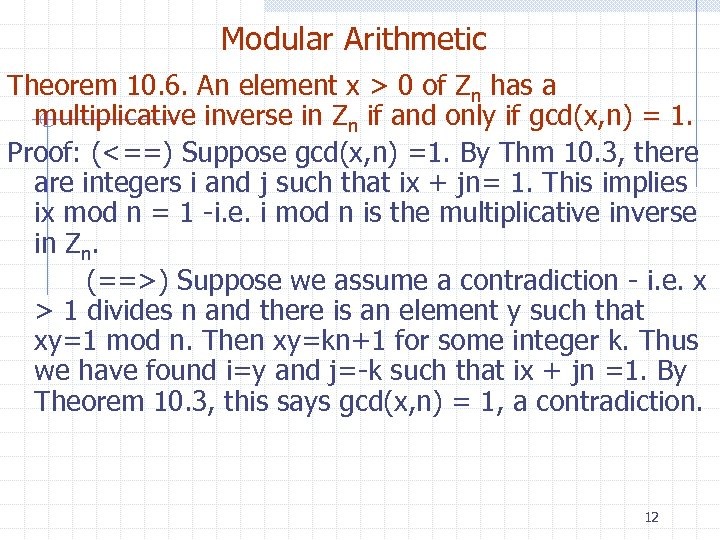Modular Arithmetic Theorem 10. 6. An element x > 0 of Zn has a multiplicative inverse in Zn if and only if gcd(x, n) = 1. Proof: (<==) Suppose gcd(x, n) =1. By Thm 10. 3, there are integers i and j such that ix + jn= 1. This implies ix mod n = 1 -i. e. i mod n is the multiplicative inverse in Zn. (==>) Suppose we assume a contradiction - i. e. x > 1 divides n and there is an element y such that xy=1 mod n. Then xy=kn+1 for some integer k. Thus we have found i=y and j=-k such that ix + jn =1. By Theorem 10. 3, this says gcd(x, n) = 1, a contradiction. 12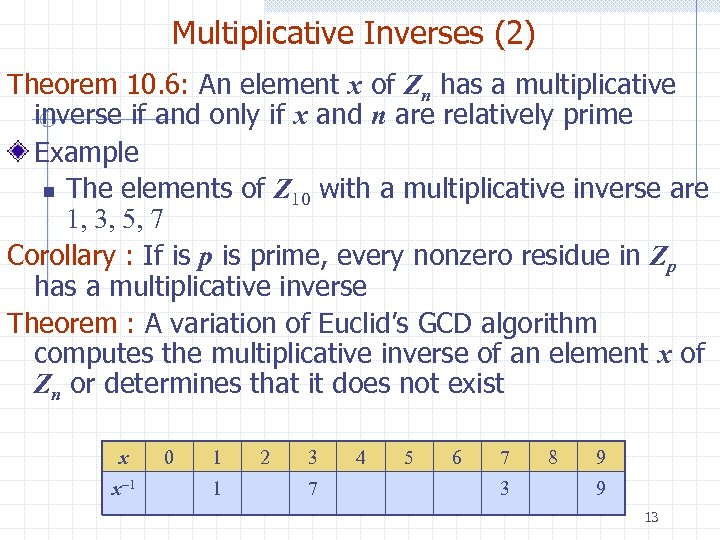Multiplicative Inverses (2) Theorem 10. 6: An element x of Zn has a multiplicative inverse if and only if x and n are relatively prime Example n The elements of Z 10 with a multiplicative inverse are 1, 3, 5, 7 Corollary : If is prime, every nonzero residue in Zp has a multiplicative inverse Theorem : A variation of Euclid’s GCD algorithm computes the multiplicative inverse of an element x of Zn or determines that it does not exist x x-1 0 1 1 2 3 7 4 5 6 7 3 8 9 9 13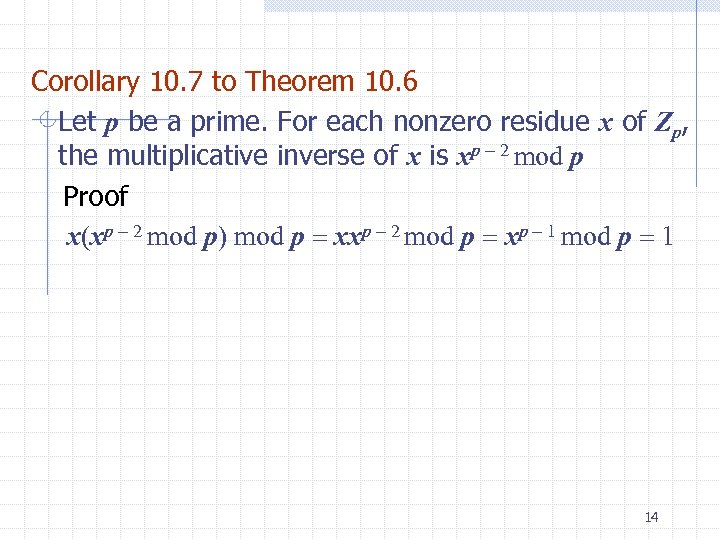Corollary 10. 7 to Theorem 10. 6 Let p be a prime. For each nonzero residue x of Zp, the multiplicative inverse of x is xp - 2 mod p Proof x(xp - 2 mod p) mod p = xxp - 2 mod p = xp - 1 mod p = 1 14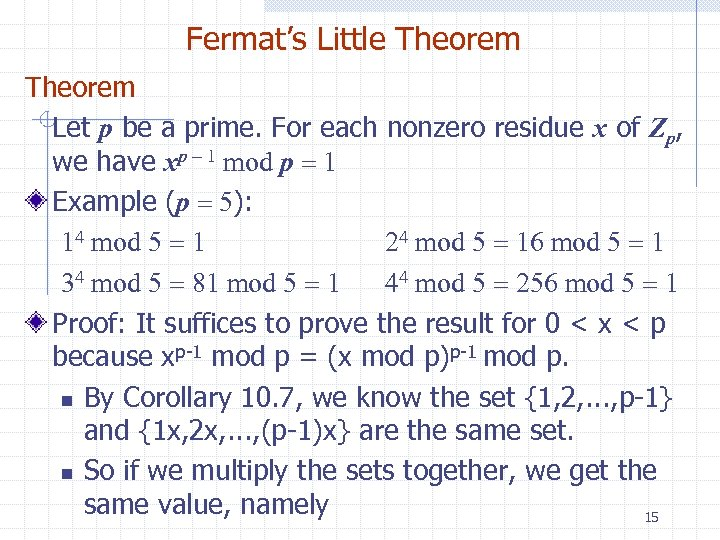Fermat’s Little Theorem Let p be a prime. For each nonzero residue x of Zp, we have xp - 1 mod p = 1 Example (p = 5): 14 mod 5 = 1 24 mod 5 = 16 mod 5 = 1 34 mod 5 = 81 mod 5 = 1 44 mod 5 = 256 mod 5 = 1 Proof: It suffices to prove the result for 0 < x < p because xp-1 mod p = (x mod p)p-1 mod p. n By Corollary 10. 7, we know the set {1, 2, . . . , p-1} and {1 x, 2 x, . . . , (p-1)x} are the same set. n So if we multiply the sets together, we get the same value, namely 15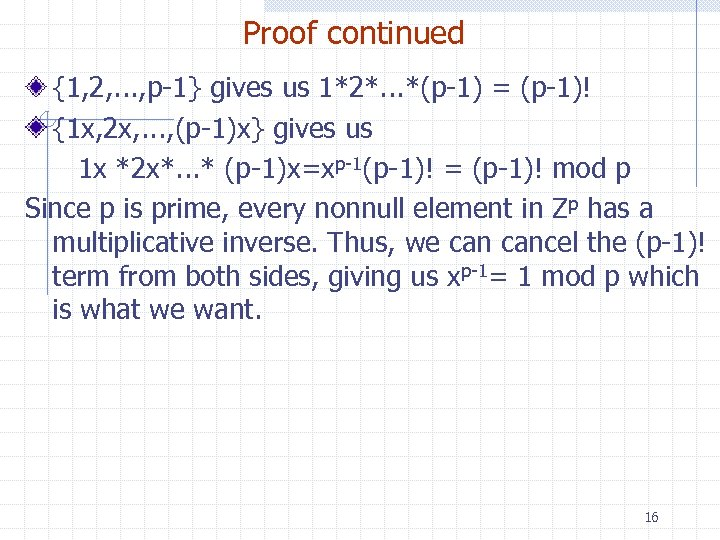Proof continued {1, 2, . . . , p-1} gives us 1*2*. . . *(p-1) = (p-1)! {1 x, 2 x, . . . , (p-1)x} gives us 1 x *2 x*. . . * (p-1)x=xp-1(p-1)! = (p-1)! mod p Since p is prime, every nonnull element in Zp has a multiplicative inverse. Thus, we cancel the (p-1)! term from both sides, giving us xp-1= 1 mod p which is what we want. 16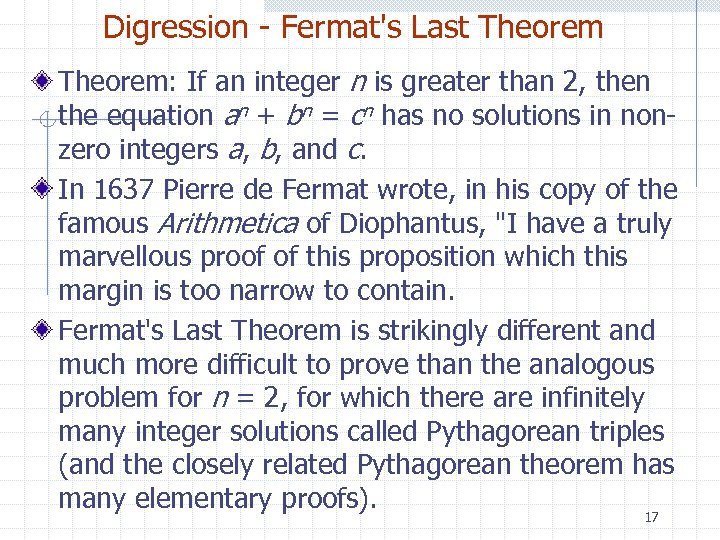Digression - Fermat's Last Theorem: If an integer n is greater than 2, then the equation an + bn = cn has no solutions in nonzero integers a, b, and c. In 1637 Pierre de Fermat wrote, in his copy of the famous Arithmetica of Diophantus, "I have a truly marvellous proof of this proposition which this margin is too narrow to contain. Fermat's Last Theorem is strikingly different and much more difficult to prove than the analogous problem for n = 2, for which there are infinitely many integer solutions called Pythagorean triples (and the closely related Pythagorean theorem has many elementary proofs). 17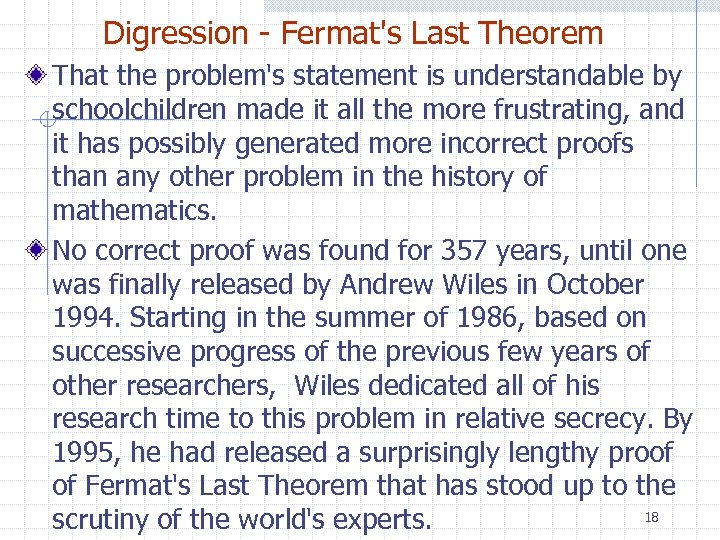Digression - Fermat's Last Theorem That the problem's statement is understandable by schoolchildren made it all the more frustrating, and it has possibly generated more incorrect proofs than any other problem in the history of mathematics. No correct proof was found for 357 years, until one was finally released by Andrew Wiles in October 1994. Starting in the summer of 1986, based on successive progress of the previous few years of other researchers, Wiles dedicated all of his research time to this problem in relative secrecy. By 1995, he had released a surprisingly lengthy proof of Fermat's Last Theorem that has stood up to the 18 scrutiny of the world's experts.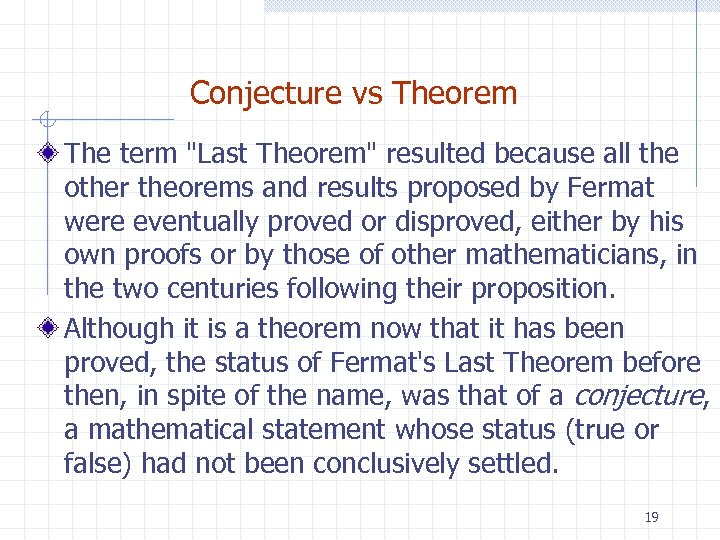Conjecture vs Theorem The term "Last Theorem" resulted because all the other theorems and results proposed by Fermat were eventually proved or disproved, either by his own proofs or by those of other mathematicians, in the two centuries following their proposition. Although it is a theorem now that it has been proved, the status of Fermat's Last Theorem before then, in spite of the name, was that of a conjecture, a mathematical statement whose status (true or false) had not been conclusively settled. 19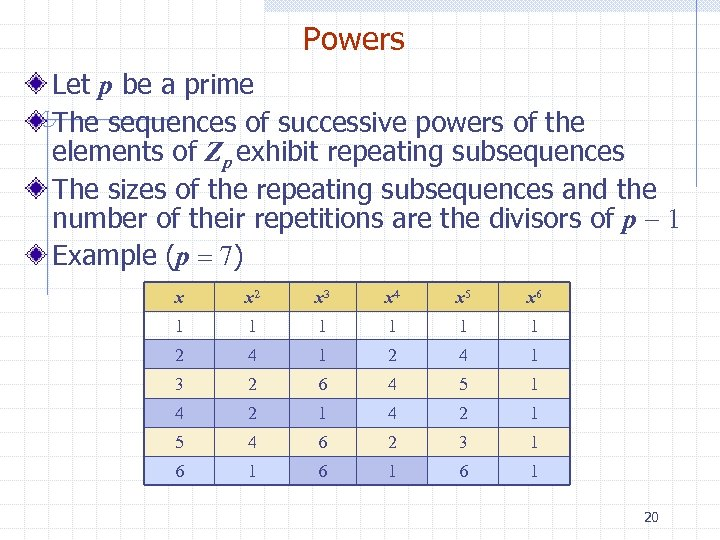Powers Let p be a prime The sequences of successive powers of the elements of Zp exhibit repeating subsequences The sizes of the repeating subsequences and the number of their repetitions are the divisors of p - 1 Example (p = 7) x x 2 x 3 x 4 x 5 x 6 1 1 1 2 4 1 3 2 6 4 5 1 4 2 1 5 4 6 2 3 1 6 1 6 1 20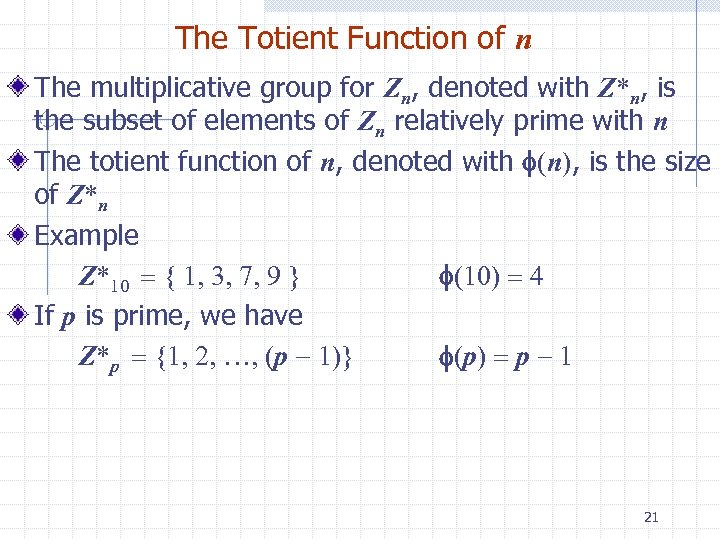The Totient Function of n The multiplicative group for Zn, denoted with Z*n, is the subset of elements of Zn relatively prime with n The totient function of n, denoted with f(n), is the size of Z*n Example Z*10 = { 1, 3, 7, 9 } f(10) = 4 If p is prime, we have Z*p = {1, 2, …, (p - 1)} f(p) = p - 1 21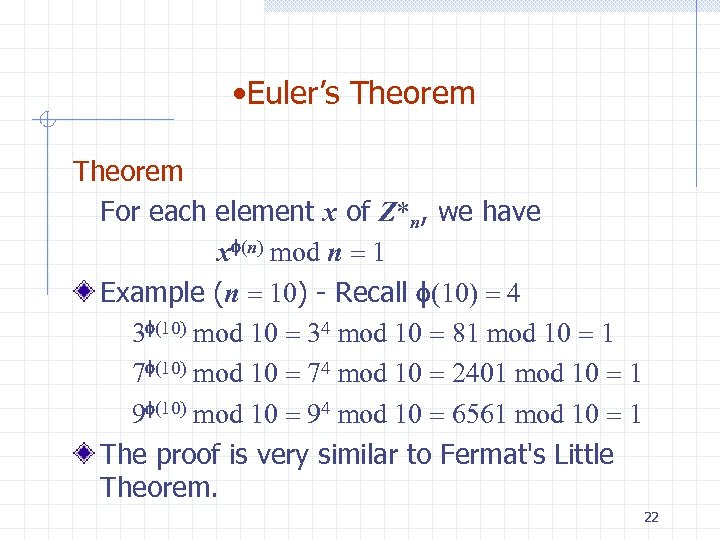• Euler’s Theorem For each element x of Z*n, we have xf(n) mod n = 1 Example (n = 10) - Recall f(10) = 4 3 f(10) mod 10 = 34 mod 10 = 81 mod 10 = 1 7 f(10) mod 10 = 74 mod 10 = 2401 mod 10 = 1 9 f(10) mod 10 = 94 mod 10 = 6561 mod 10 = 1 The proof is very similar to Fermat's Little Theorem. 22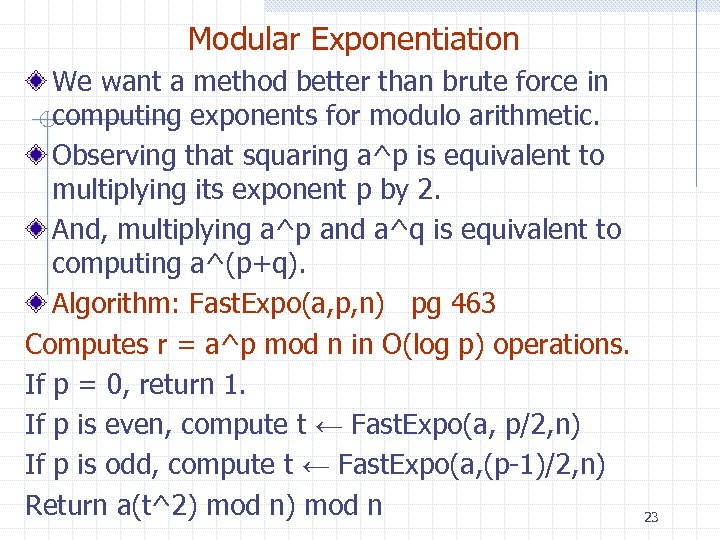Modular Exponentiation We want a method better than brute force in computing exponents for modulo arithmetic. Observing that squaring a^p is equivalent to multiplying its exponent p by 2. And, multiplying a^p and a^q is equivalent to computing a^(p+q). Algorithm: Fast. Expo(a, p, n) pg 463 Computes r = a^p mod n in O(log p) operations. If p = 0, return 1. If p is even, compute t ← Fast. Expo(a, p/2, n) If p is odd, compute t ← Fast. Expo(a, (p-1)/2, n) Return a(t^2) mod n 23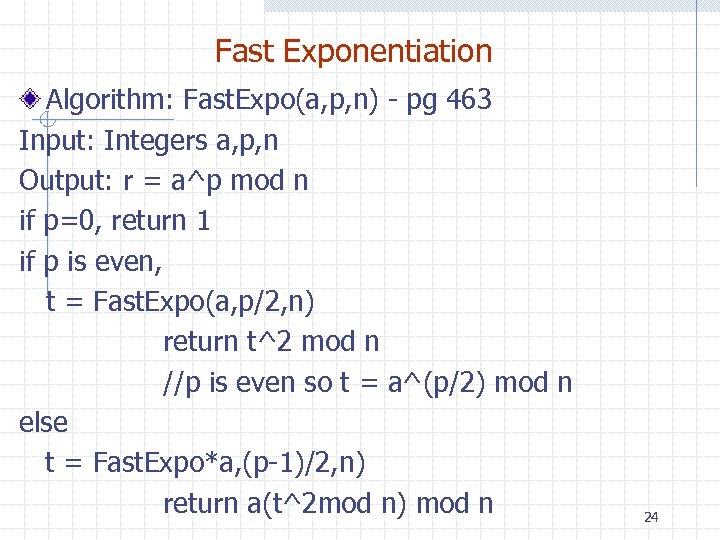Fast Exponentiation Algorithm: Fast. Expo(a, p, n) - pg 463 Input: Integers a, p, n Output: r = a^p mod n if p=0, return 1 if p is even, t = Fast. Expo(a, p/2, n) return t^2 mod n //p is even so t = a^(p/2) mod n else t = Fast. Expo*a, (p-1)/2, n) return a(t^2 mod n) mod n 24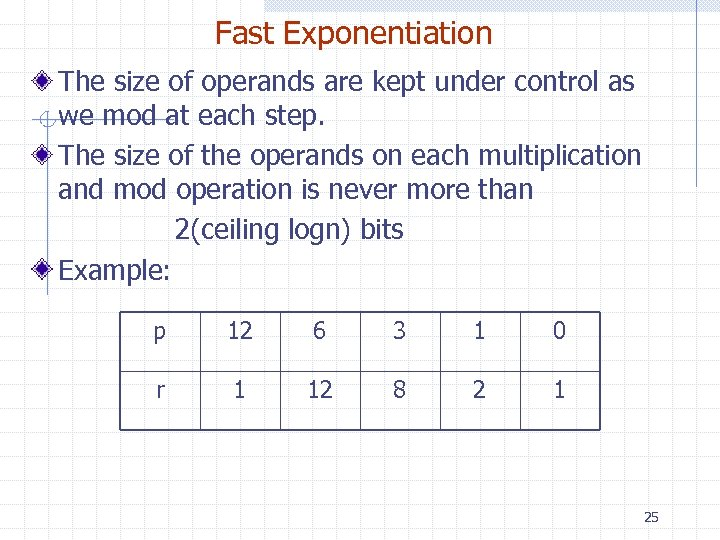Fast Exponentiation The size of operands are kept under control as we mod at each step. The size of the operands on each multiplication and mod operation is never more than 2(ceiling logn) bits Example: p 12 6 3 1 0 r 1 12 8 2 1 25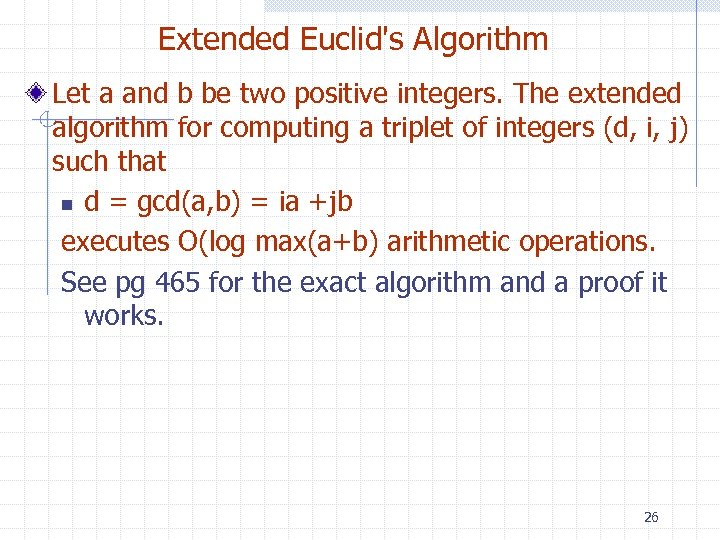Extended Euclid's Algorithm Let a and b be two positive integers. The extended algorithm for computing a triplet of integers (d, i, j) such that n d = gcd(a, b) = ia +jb executes O(log max(a+b) arithmetic operations. See pg 465 for the exact algorithm and a proof it works. 26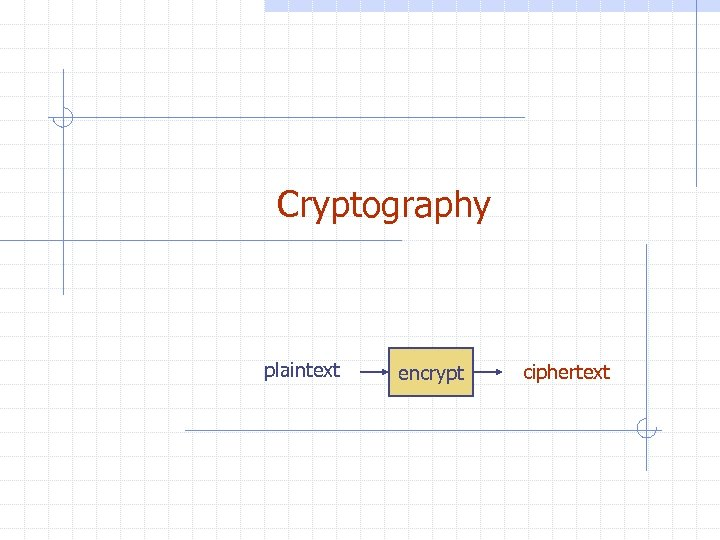Cryptography plaintext encrypt ciphertext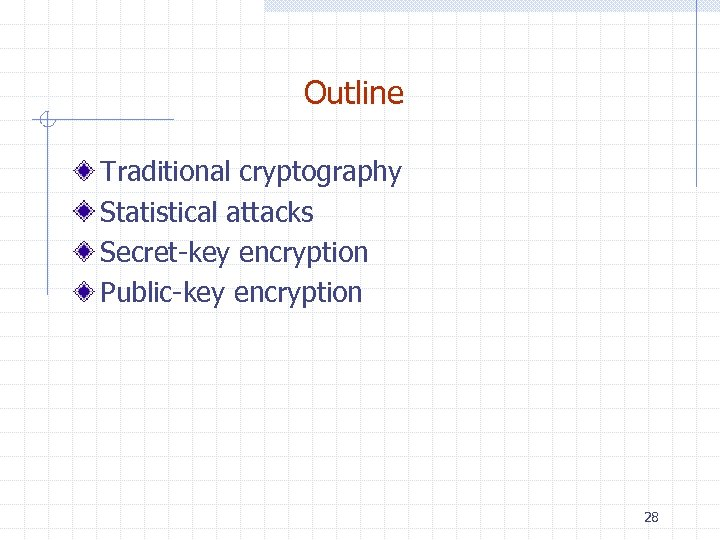Outline Traditional cryptography Statistical attacks Secret-key encryption Public-key encryption 28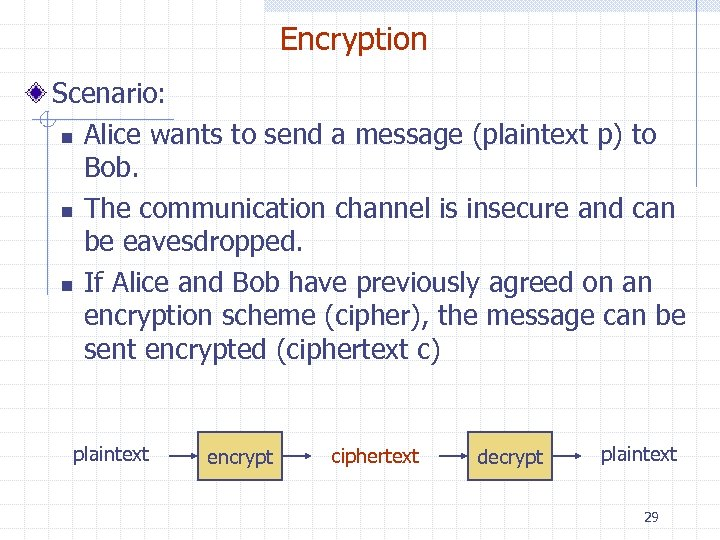Encryption Scenario: n Alice wants to send a message (plaintext p) to Bob. n The communication channel is insecure and can be eavesdropped. n If Alice and Bob have previously agreed on an encryption scheme (cipher), the message can be sent encrypted (ciphertext c) plaintext encrypt ciphertext decrypt plaintext 29Encryption Issues: n What is a good encryption scheme? n What is the complexity of encrypting/decrypting? n What is the size of the ciphertext, relative to the plaintext? n If Alice and Bob have never interacted before, how can they agree on an encryption scheme? plaintext encrypt ciphertext decrypt plaintext 30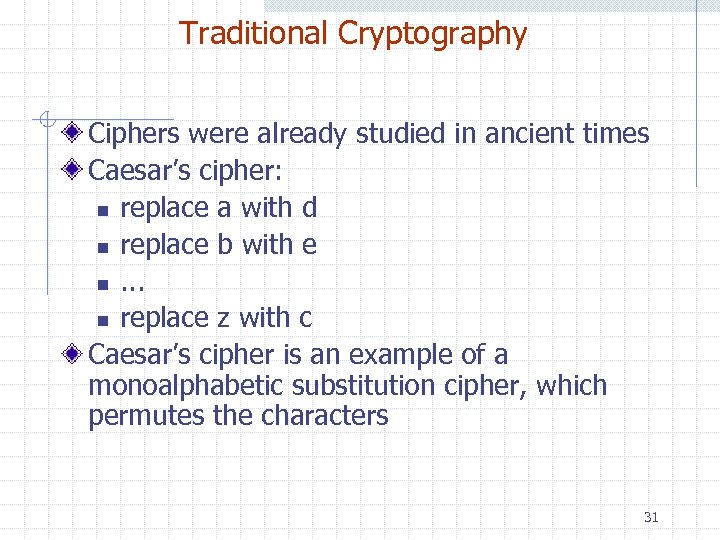Traditional Cryptography Ciphers were already studied in ancient times Caesar’s cipher: n replace a with d n replace b with e n. . . n replace z with c Caesar’s cipher is an example of a monoalphabetic substitution cipher, which permutes the characters 31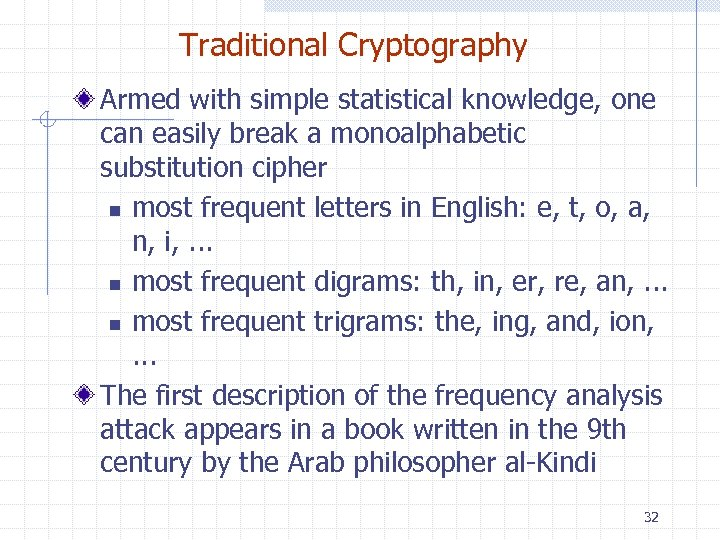Traditional Cryptography Armed with simple statistical knowledge, one can easily break a monoalphabetic substitution cipher n most frequent letters in English: e, t, o, a, n, i, . . . n most frequent digrams: th, in, er, re, an, . . . n most frequent trigrams: the, ing, and, ion, . . . The first description of the frequency analysis attack appears in a book written in the 9 th century by the Arab philosopher al-Kindi 32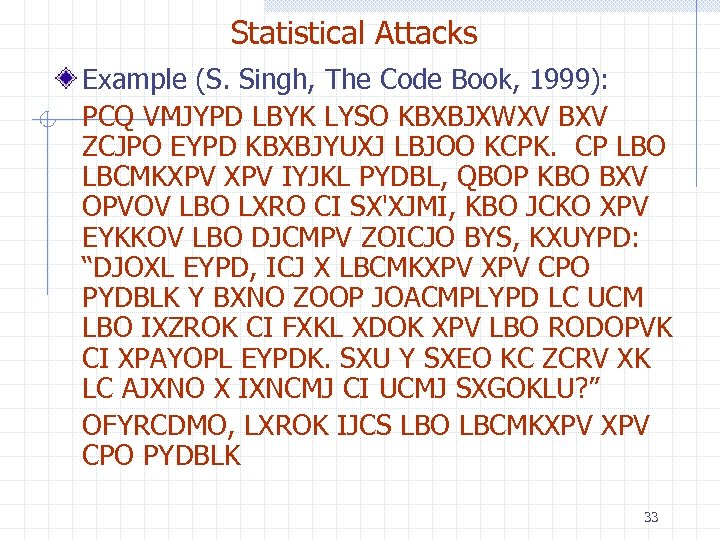Statistical Attacks Example (S. Singh, The Code Book, 1999): PCQ VMJYPD LBYK LYSO KBXBJXWXV BXV ZCJPO EYPD KBXBJYUXJ LBJOO KCPK. CP LBO LBCMKXPV IYJKL PYDBL, QBOP KBO BXV OPVOV LBO LXRO CI SX'XJMI, KBO JCKO XPV EYKKOV LBO DJCMPV ZOICJO BYS, KXUYPD: “DJOXL EYPD, ICJ X LBCMKXPV CPO PYDBLK Y BXNO ZOOP JOACMPLYPD LC UCM LBO IXZROK CI FXKL XDOK XPV LBO RODOPVK CI XPAYOPL EYPDK. SXU Y SXEO KC ZCRV XK LC AJXNO X IXNCMJ CI UCMJ SXGOKLU? ” OFYRCDMO, LXROK IJCS LBO LBCMKXPV CPO PYDBLK 33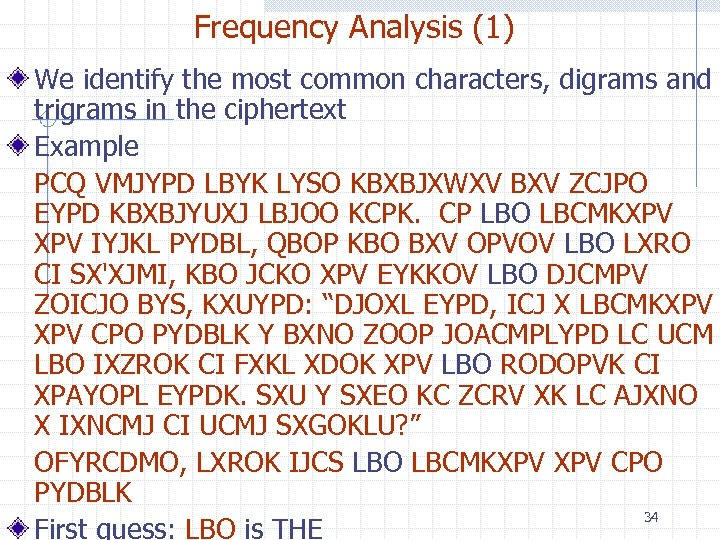Frequency Analysis (1) We identify the most common characters, digrams and trigrams in the ciphertext Example PCQ VMJYPD LBYK LYSO KBXBJXWXV BXV ZCJPO EYPD KBXBJYUXJ LBJOO KCPK. CP LBO LBCMKXPV IYJKL PYDBL, QBOP KBO BXV OPVOV LBO LXRO CI SX'XJMI, KBO JCKO XPV EYKKOV LBO DJCMPV ZOICJO BYS, KXUYPD: “DJOXL EYPD, ICJ X LBCMKXPV CPO PYDBLK Y BXNO ZOOP JOACMPLYPD LC UCM LBO IXZROK CI FXKL XDOK XPV LBO RODOPVK CI XPAYOPL EYPDK. SXU Y SXEO KC ZCRV XK LC AJXNO X IXNCMJ CI UCMJ SXGOKLU? ” OFYRCDMO, LXROK IJCS LBO LBCMKXPV CPO PYDBLK 34 First guess: LBO is THE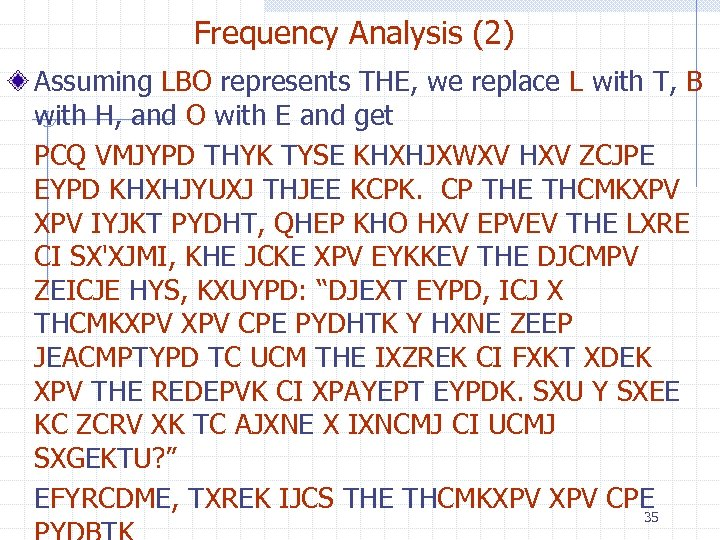Frequency Analysis (2) Assuming LBO represents THE, we replace L with T, B with H, and O with E and get PCQ VMJYPD THYK TYSE KHXHJXWXV HXV ZCJPE EYPD KHXHJYUXJ THJEE KCPK. CP THE THCMKXPV IYJKT PYDHT, QHEP KHO HXV EPVEV THE LXRE CI SX'XJMI, KHE JCKE XPV EYKKEV THE DJCMPV ZEICJE HYS, KXUYPD: “DJEXT EYPD, ICJ X THCMKXPV CPE PYDHTK Y HXNE ZEEP JEACMPTYPD TC UCM THE IXZREK CI FXKT XDEK XPV THE REDEPVK CI XPAYEPT EYPDK. SXU Y SXEE KC ZCRV XK TC AJXNE X IXNCMJ CI UCMJ SXGEKTU? ” EFYRCDME, TXREK IJCS THE THCMKXPV CPE 35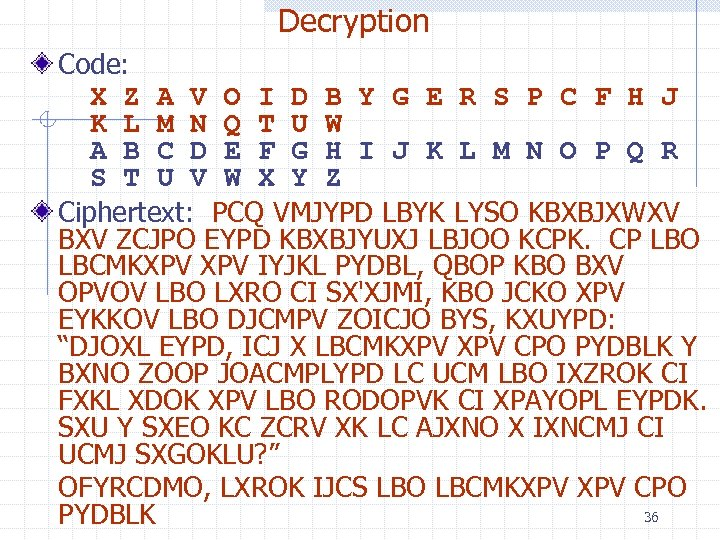Decryption Code: X Z A V O I D B Y G E R S P C F H J K L M N Q T U W A B C D E F G H I J K L M N O P Q R S T U V W X Y Z Ciphertext: PCQ VMJYPD LBYK LYSO KBXBJXWXV BXV ZCJPO EYPD KBXBJYUXJ LBJOO KCPK. CP LBO LBCMKXPV IYJKL PYDBL, QBOP KBO BXV OPVOV LBO LXRO CI SX'XJMI, KBO JCKO XPV EYKKOV LBO DJCMPV ZOICJO BYS, KXUYPD: “DJOXL EYPD, ICJ X LBCMKXPV CPO PYDBLK Y BXNO ZOOP JOACMPLYPD LC UCM LBO IXZROK CI FXKL XDOK XPV LBO RODOPVK CI XPAYOPL EYPDK. SXU Y SXEO KC ZCRV XK LC AJXNO X IXNCMJ CI UCMJ SXGOKLU? ” OFYRCDMO, LXROK IJCS LBO LBCMKXPV CPO 36 PYDBLK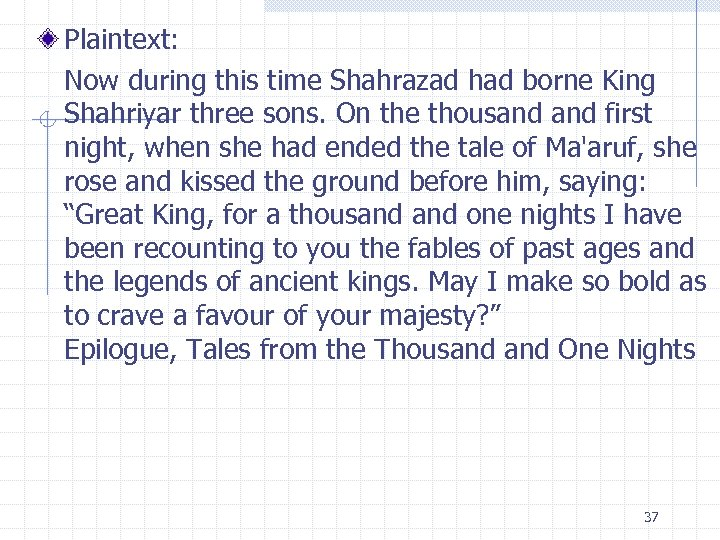Plaintext: Now during this time Shahrazad had borne King Shahriyar three sons. On the thousand first night, when she had ended the tale of Ma'aruf, she rose and kissed the ground before him, saying: “Great King, for a thousand one nights I have been recounting to you the fables of past ages and the legends of ancient kings. May I make so bold as to crave a favour of your majesty? ” Epilogue, Tales from the Thousand One Nights 37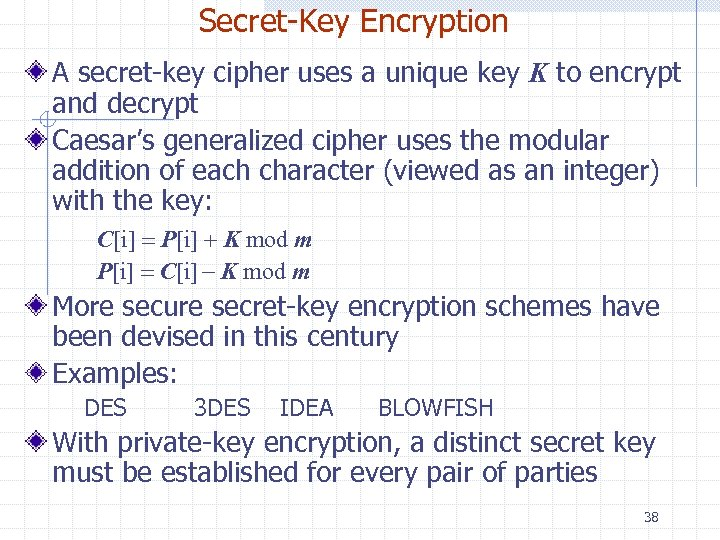Secret-Key Encryption A secret-key cipher uses a unique key K to encrypt and decrypt Caesar’s generalized cipher uses the modular addition of each character (viewed as an integer) with the key: C[i] = P[i] + K mod m P[i] = C[i] - K mod m More secure secret-key encryption schemes have been devised in this century Examples: DES 3 DES IDEA BLOWFISH With private-key encryption, a distinct secret key must be established for every pair of parties 38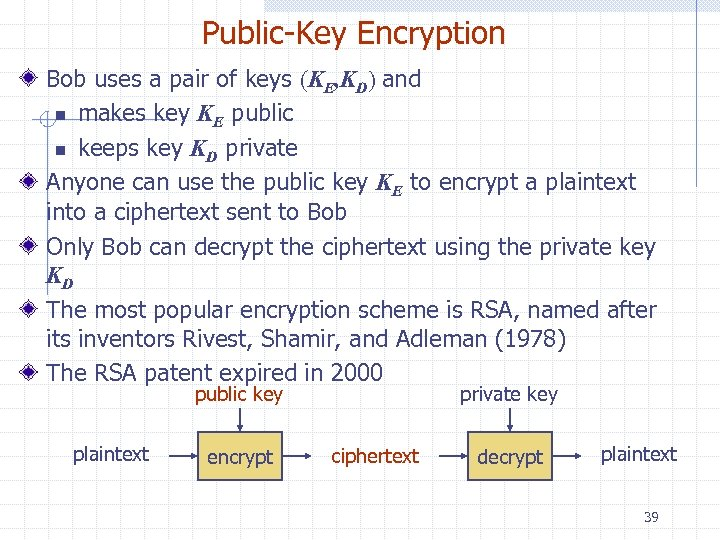Public-Key Encryption Bob uses a pair of keys (KE, KD) and n makes key KE public n keeps key KD private Anyone can use the public key KE to encrypt a plaintext into a ciphertext sent to Bob Only Bob can decrypt the ciphertext using the private key KD The most popular encryption scheme is RSA, named after its inventors Rivest, Shamir, and Adleman (1978) The RSA patent expired in 2000 public key plaintext encrypt private key ciphertext decrypt plaintext 39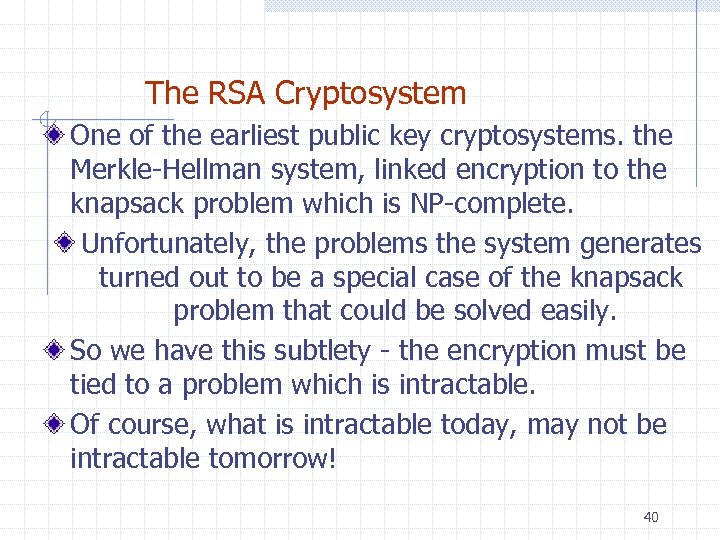The RSA Cryptosystem One of the earliest public key cryptosystems. the Merkle-Hellman system, linked encryption to the knapsack problem which is NP-complete. Unfortunately, the problems the system generates turned out to be a special case of the knapsack problem that could be solved easily. So we have this subtlety - the encryption must be tied to a problem which is intractable. Of course, what is intractable today, may not be intractable tomorrow! 40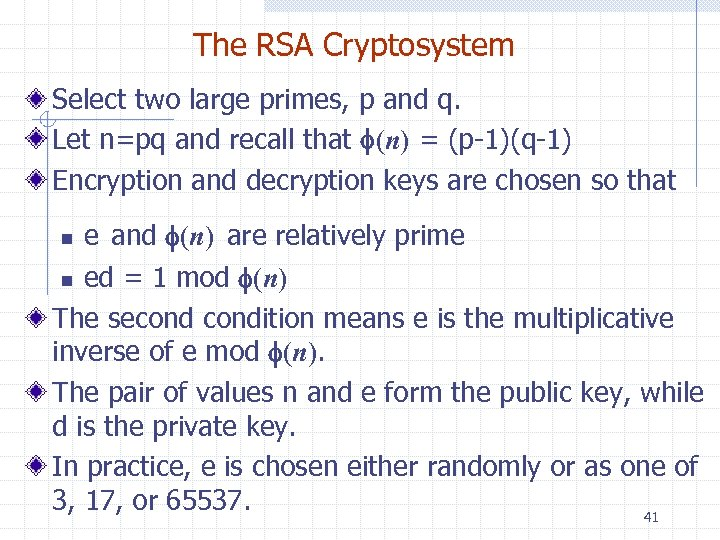The RSA Cryptosystem Select two large primes, p and q. Let n=pq and recall that f(n) = (p-1)(q-1) Encryption and decryption keys are chosen so that e and f(n) are relatively prime n ed = 1 mod f(n) The secondition means e is the multiplicative inverse of e mod f(n). The pair of values n and e form the public key, while d is the private key. In practice, e is chosen either randomly or as one of 3, 17, or 65537. 41 n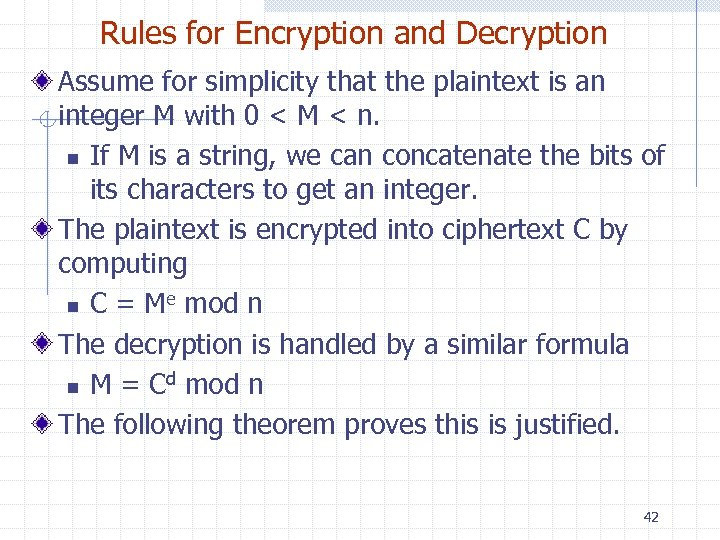Rules for Encryption and Decryption Assume for simplicity that the plaintext is an integer M with 0 < M < n. n If M is a string, we can concatenate the bits of its characters to get an integer. The plaintext is encrypted into ciphertext C by computing n C = Me mod n The decryption is handled by a similar formula n M = Cd mod n The following theorem proves this is justified. 42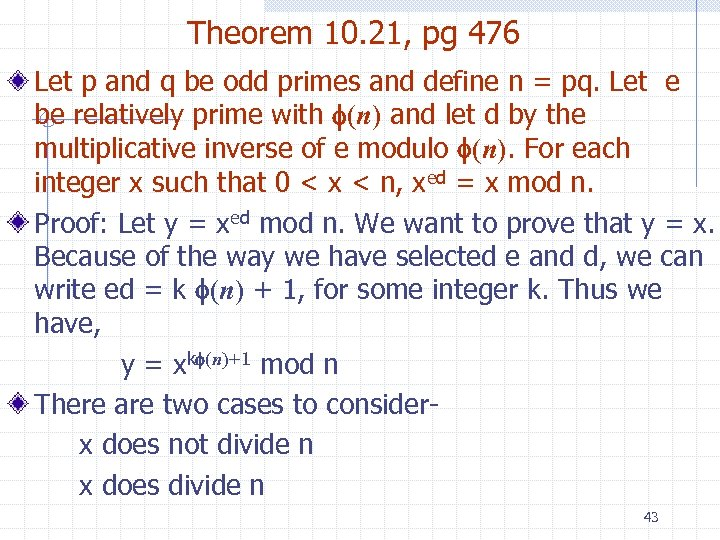Theorem 10. 21, pg 476 Let p and q be odd primes and define n = pq. Let e be relatively prime with f(n) and let d by the multiplicative inverse of e modulo f(n). For each integer x such that 0 < x < n, xed = x mod n. Proof: Let y = xed mod n. We want to prove that y = x. Because of the way we have selected e and d, we can write ed = k f(n) + 1, for some integer k. Thus we have, y = xkf(n)+1 mod n There are two cases to considerx does not divide n x does divide n 43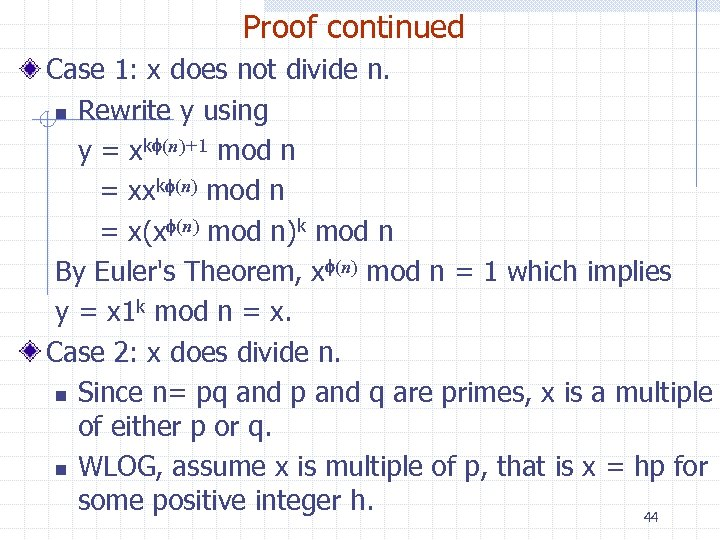Proof continued Case 1: x does not divide n. n Rewrite y using y = xkf(n)+1 mod n = xxkf(n) mod n = x(xf(n) mod n)k mod n By Euler's Theorem, xf(n) mod n = 1 which implies y = x 1 k mod n = x. Case 2: x does divide n. n Since n= pq and p and q are primes, x is a multiple of either p or q. n WLOG, assume x is multiple of p, that is x = hp for some positive integer h. 44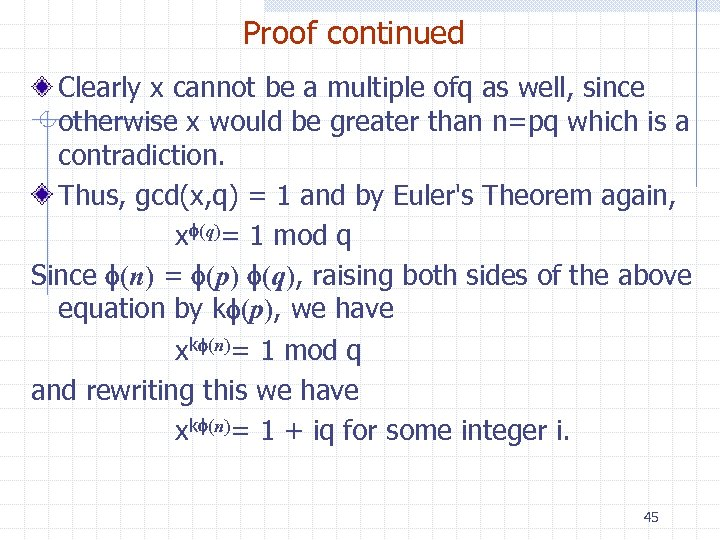Proof continued Clearly x cannot be a multiple ofq as well, since otherwise x would be greater than n=pq which is a contradiction. Thus, gcd(x, q) = 1 and by Euler's Theorem again, xf(q)= 1 mod q Since f(n) = f(p) f(q), raising both sides of the above equation by kf(p), we have xkf(n)= 1 mod q and rewriting this we have xkf(n)= 1 + iq for some integer i. 45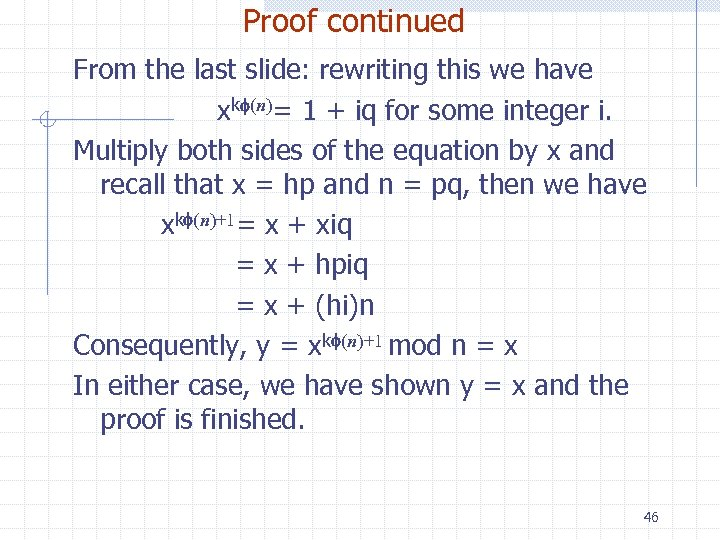Proof continued From the last slide: rewriting this we have xkf(n)= 1 + iq for some integer i. Multiply both sides of the equation by x and recall that x = hp and n = pq, then we have xkf(n)+1= x + xiq = x + hpiq = x + (hi)n Consequently, y = xkf(n)+1 mod n = x In either case, we have shown y = x and the proof is finished. 46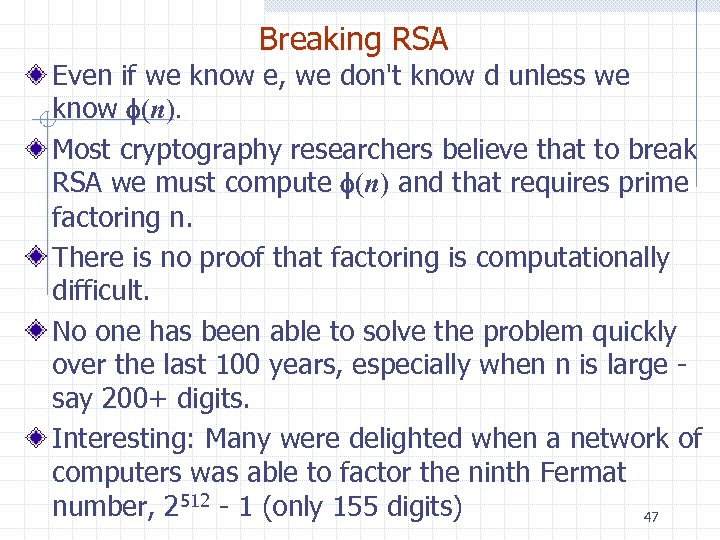Breaking RSA Even if we know e, we don't know d unless we know f(n). Most cryptography researchers believe that to break RSA we must compute f(n) and that requires prime factoring n. There is no proof that factoring is computationally difficult. No one has been able to solve the problem quickly over the last 100 years, especially when n is large - say 200+ digits. Interesting: Many were delighted when a network of computers was able to factor the ninth Fermat number, 2512 - 1 (only 155 digits) 47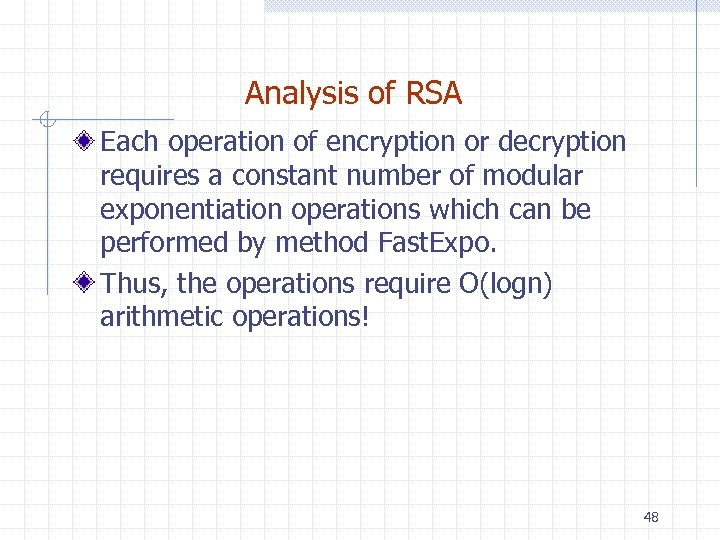Analysis of RSA Each operation of encryption or decryption requires a constant number of modular exponentiation operations which can be performed by method Fast. Expo. Thus, the operations require O(logn) arithmetic operations! 48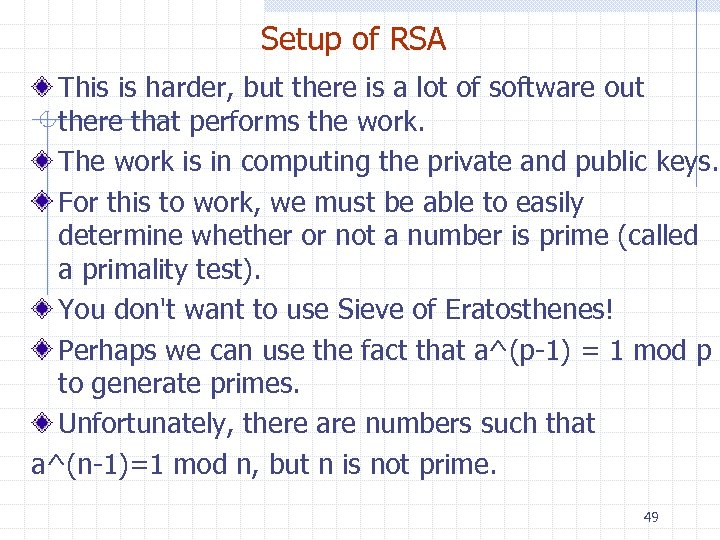Setup of RSA This is harder, but there is a lot of software out there that performs the work. The work is in computing the private and public keys. For this to work, we must be able to easily determine whether or not a number is prime (called a primality test). You don't want to use Sieve of Eratosthenes! Perhaps we can use the fact that a^(p-1) = 1 mod p to generate primes. Unfortunately, there are numbers such that a^(n-1)=1 mod n, but n is not prime. 49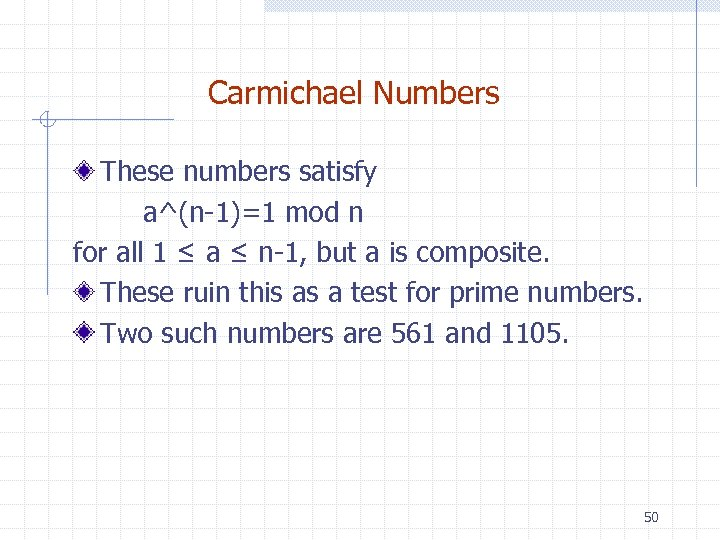Carmichael Numbers These numbers satisfy a^(n-1)=1 mod n for all 1 ≤ a ≤ n-1, but a is composite. These ruin this as a test for prime numbers. Two such numbers are 561 and 1105. 50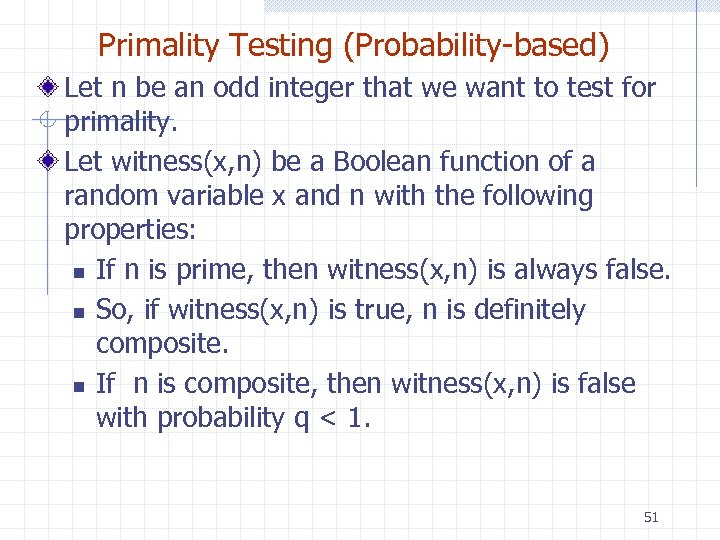Primality Testing (Probability-based) Let n be an odd integer that we want to test for primality. Let witness(x, n) be a Boolean function of a random variable x and n with the following properties: n If n is prime, then witness(x, n) is always false. n So, if witness(x, n) is true, n is definitely composite. n If n is composite, then witness(x, n) is false with probability q < 1. 51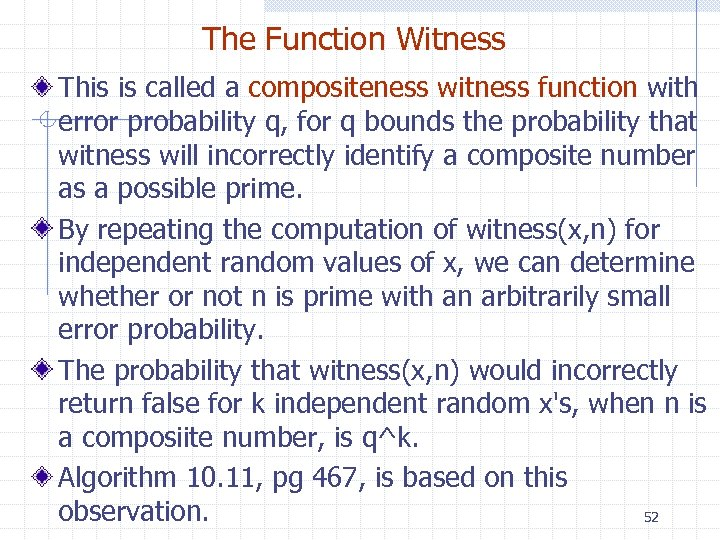The Function Witness This is called a compositeness witness function with error probability q, for q bounds the probability that witness will incorrectly identify a composite number as a possible prime. By repeating the computation of witness(x, n) for independent random values of x, we can determine whether or not n is prime with an arbitrarily small error probability. The probability that witness(x, n) would incorrectly return false for k independent random x's, when n is a composiite number, is q^k. Algorithm 10. 11, pg 467, is based on this observation. 52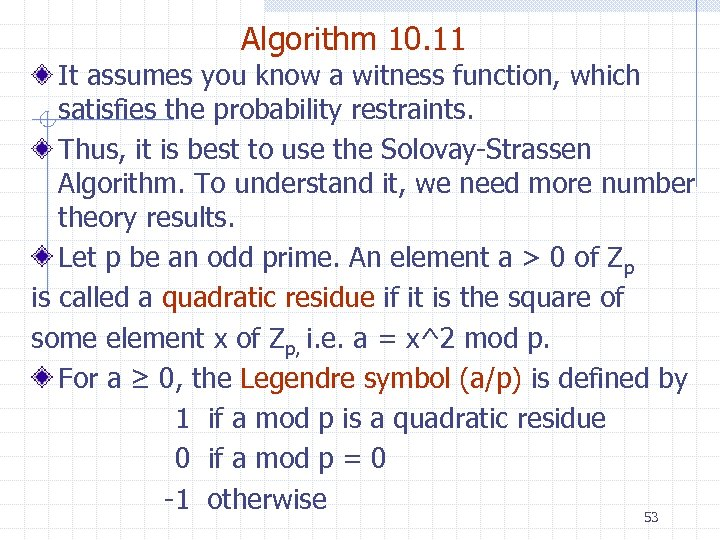Algorithm 10. 11 It assumes you know a witness function, which satisfies the probability restraints. Thus, it is best to use the Solovay-Strassen Algorithm. To understand it, we need more number theory results. Let p be an odd prime. An element a > 0 of Zp is called a quadratic residue if it is the square of some element x of Zp, i. e. a = x^2 mod p. For a ≥ 0, the Legendre symbol (a/p) is defined by 1 if a mod p is a quadratic residue 0 if a mod p = 0 -1 otherwise 53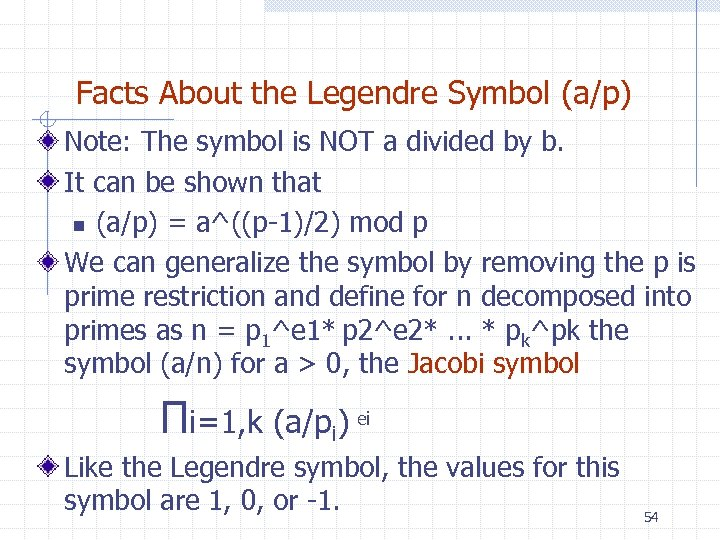Facts About the Legendre Symbol (a/p) Note: The symbol is NOT a divided by b. It can be shown that n (a/p) = a^((p-1)/2) mod p We can generalize the symbol by removing the p is prime restriction and define for n decomposed into primes as n = p 1^e 1* p 2^e 2*. . . * pk^pk the symbol (a/n) for a > 0, the Jacobi symbol Πi=1, k (a/pi) ei Like the Legendre symbol, the values for this symbol are 1, 0, or -1. 54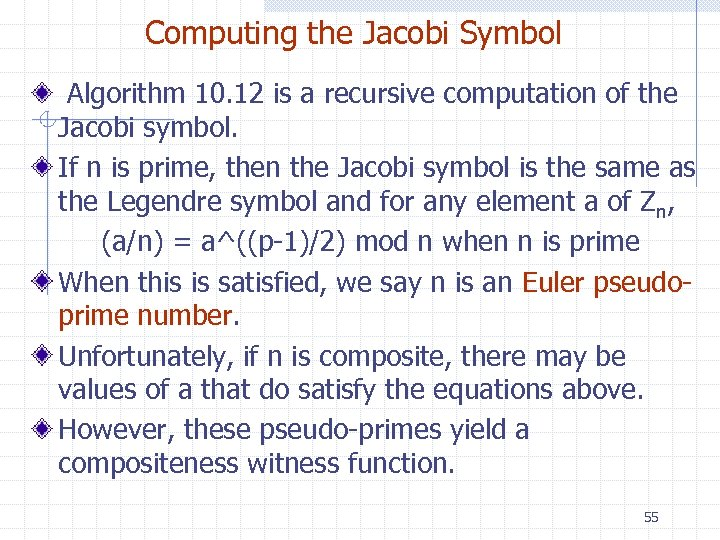Computing the Jacobi Symbol Algorithm 10. 12 is a recursive computation of the Jacobi symbol. If n is prime, then the Jacobi symbol is the same as the Legendre symbol and for any element a of Zn, (a/n) = a^((p-1)/2) mod n when n is prime When this is satisfied, we say n is an Euler pseudoprime number. Unfortunately, if n is composite, there may be values of a that do satisfy the equations above. However, these pseudo-primes yield a compositeness witness function. 55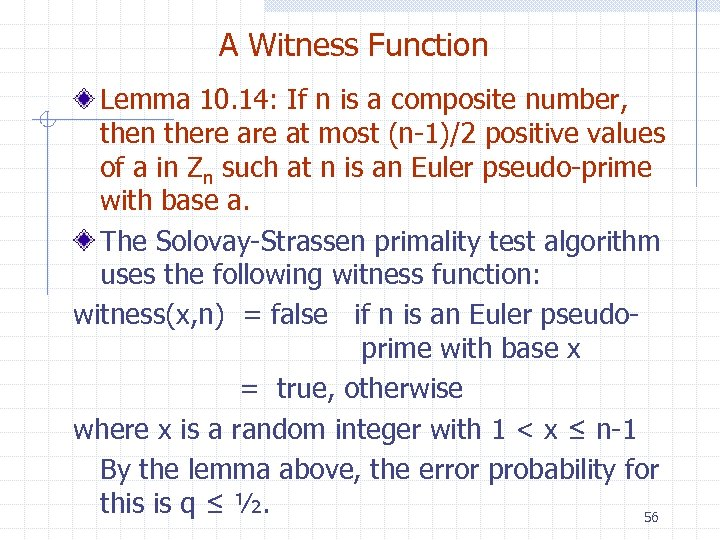A Witness Function Lemma 10. 14: If n is a composite number, then there at most (n-1)/2 positive values of a in Zn such at n is an Euler pseudo-prime with base a. The Solovay-Strassen primality test algorithm uses the following witness function: witness(x, n) = false if n is an Euler pseudoprime with base x = true, otherwise where x is a random integer with 1 < x ≤ n-1 By the lemma above, the error probability for this is q ≤ ½. 56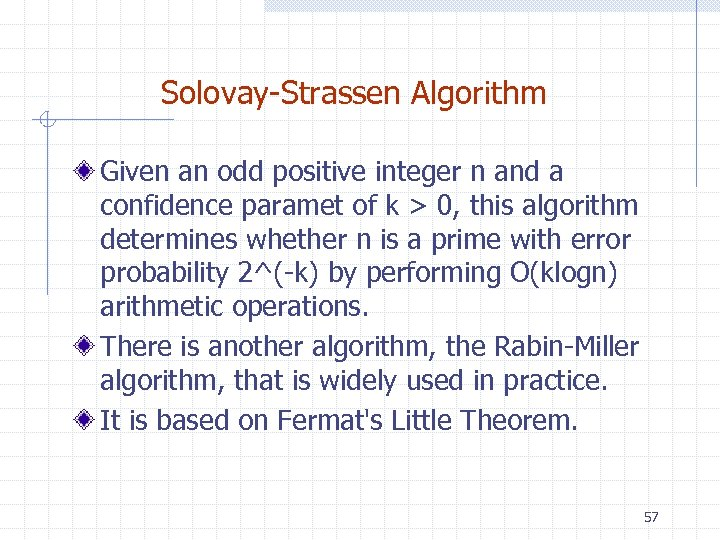Solovay-Strassen Algorithm Given an odd positive integer n and a confidence paramet of k > 0, this algorithm determines whether n is a prime with error probability 2^(-k) by performing O(klogn) arithmetic operations. There is another algorithm, the Rabin-Miller algorithm, that is widely used in practice. It is based on Fermat's Little Theorem. 57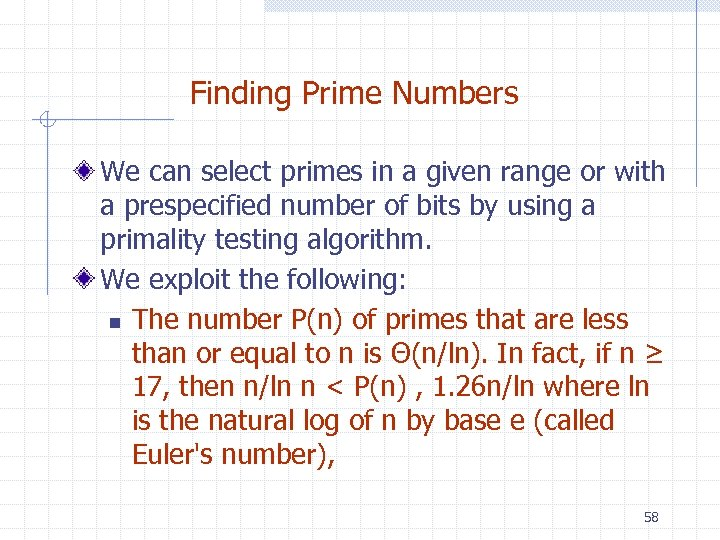Finding Prime Numbers We can select primes in a given range or with a prespecified number of bits by using a primality testing algorithm. We exploit the following: n The number P(n) of primes that are less than or equal to n is Θ(n/ln). In fact, if n ≥ 17, then n/ln n < P(n) , 1. 26 n/ln where ln is the natural log of n by base e (called Euler's number), 58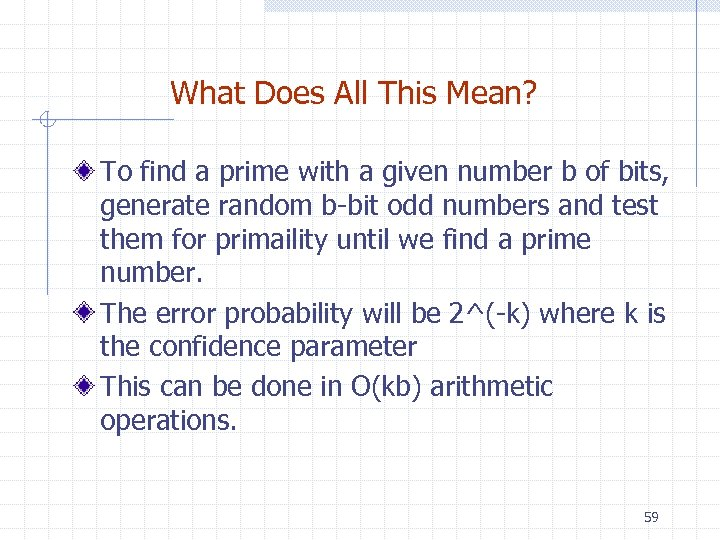What Does All This Mean? To find a prime with a given number b of bits, generate random b-bit odd numbers and test them for primaility until we find a prime number. The error probability will be 2^(-k) where k is the confidence parameter This can be done in O(kb) arithmetic operations. 59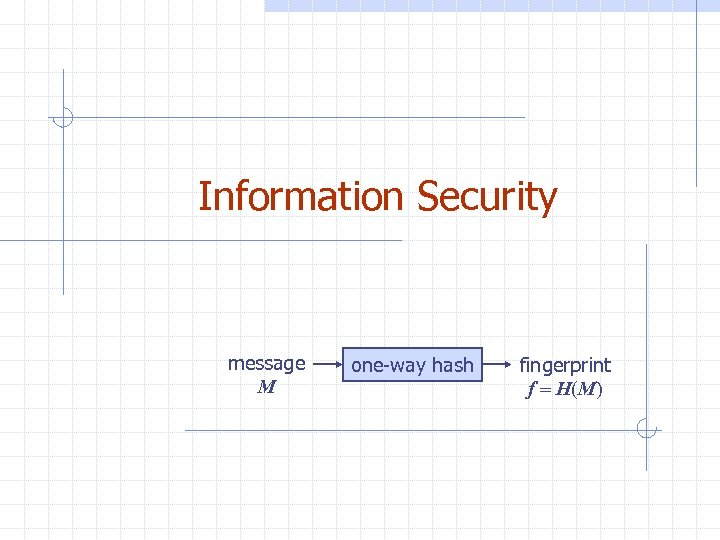Information Security message M one-way hash fingerprint f = H(M)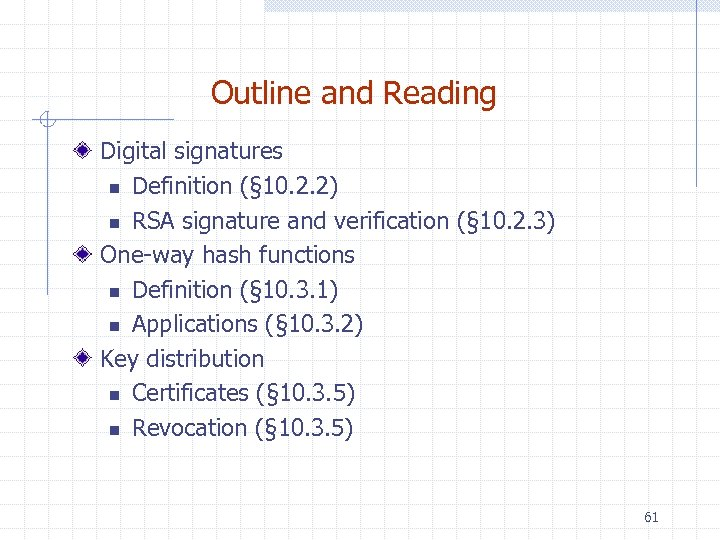Outline and Reading Digital signatures n Definition (§ 10. 2. 2) n RSA signature and verification (§ 10. 2. 3) One-way hash functions n Definition (§ 10. 3. 1) n Applications (§ 10. 3. 2) Key distribution n Certificates (§ 10. 3. 5) n Revocation (§ 10. 3. 5) 61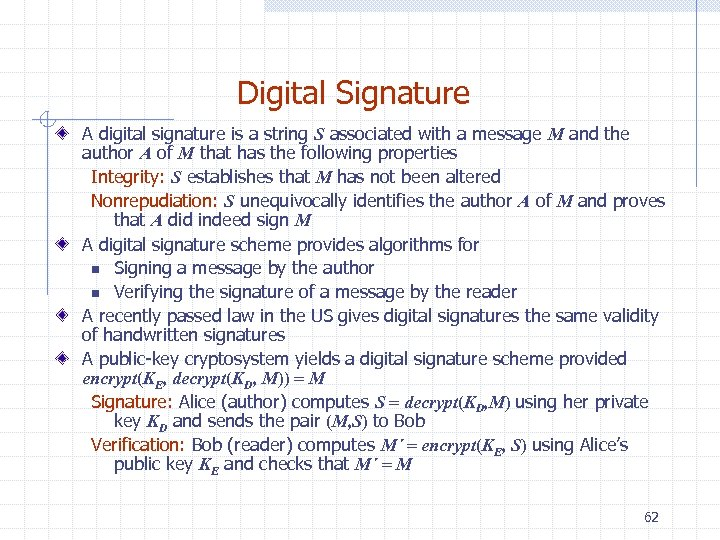Digital Signature A digital signature is a string S associated with a message M and the author A of M that has the following properties Integrity: S establishes that M has not been altered Nonrepudiation: S unequivocally identifies the author A of M and proves that A did indeed sign M A digital signature scheme provides algorithms for n Signing a message by the author n Verifying the signature of a message by the reader A recently passed law in the US gives digital signatures the same validity of handwritten signatures A public-key cryptosystem yields a digital signature scheme provided encrypt(KE, decrypt(KD, M)) = M Signature: Alice (author) computes S = decrypt(KD, M) using her private key KD and sends the pair (M, S) to Bob Verification: Bob (reader) computes M´ = encrypt(KE, S) using Alice’s public key KE and checks that M´ = M 62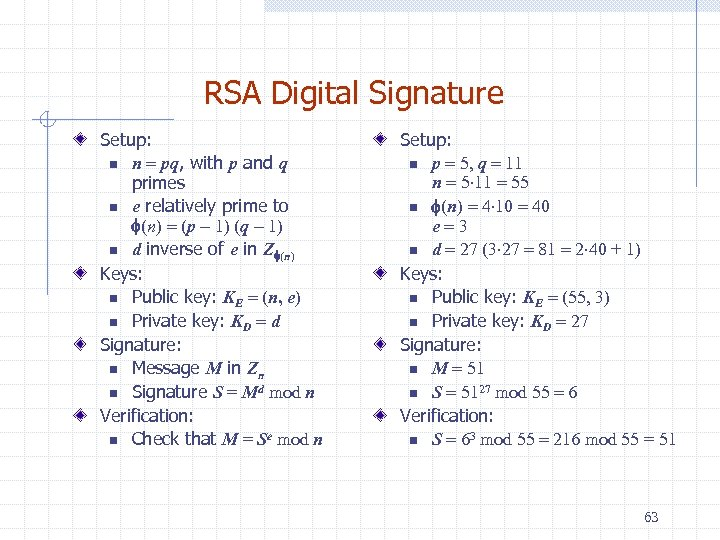RSA Digital Signature Setup: n n = pq, with p and q primes n e relatively prime to f(n) = (p - 1) (q - 1) n d inverse of e in Zf(n) Keys: n Public key: KE = (n, e) n Private key: KD = d Signature: n Message M in Zn n Signature S = Md mod n Verification: n Check that M = Se mod n Setup: n p = 5, q = 11 n = 5 11 = 55 n f(n) = 4 10 = 40 e=3 n d = 27 (3 27 = 81 = 2 40 + 1) Keys: n Public key: KE = (55, 3) n Private key: KD = 27 Signature: n M = 51 n S = 5127 mod 55 = 6 Verification: n S = 63 mod 55 = 216 mod 55 = 51 63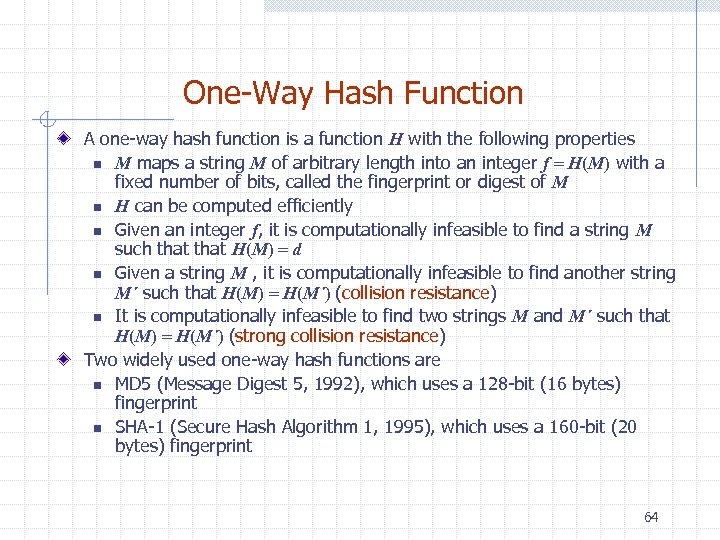One-Way Hash Function A one-way hash function is a function H with the following properties n M maps a string M of arbitrary length into an integer f = H(M) with a fixed number of bits, called the fingerprint or digest of M n H can be computed efficiently n Given an integer f, it is computationally infeasible to find a string M such that H(M) = d n Given a string M , it is computationally infeasible to find another string M´ such that H(M) = H(M´) (collision resistance) n It is computationally infeasible to find two strings M and M´ such that H(M) = H(M´) (strong collision resistance) Two widely used one-way hash functions are n MD 5 (Message Digest 5, 1992), which uses a 128 -bit (16 bytes) fingerprint n SHA-1 (Secure Hash Algorithm 1, 1995), which uses a 160 -bit (20 bytes) fingerprint 64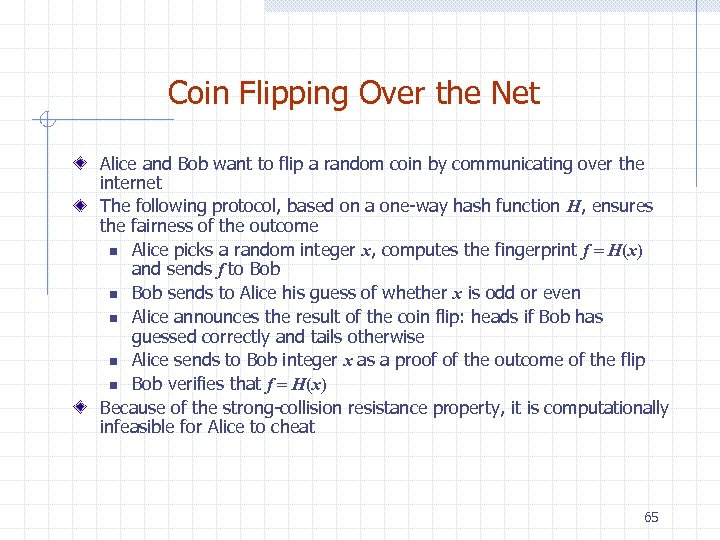Coin Flipping Over the Net Alice and Bob want to flip a random coin by communicating over the internet The following protocol, based on a one-way hash function H, ensures the fairness of the outcome n Alice picks a random integer x, computes the fingerprint f = H(x) and sends f to Bob n Bob sends to Alice his guess of whether x is odd or even n Alice announces the result of the coin flip: heads if Bob has guessed correctly and tails otherwise n Alice sends to Bob integer x as a proof of the outcome of the flip n Bob verifies that f = H(x) Because of the strong-collision resistance property, it is computationally infeasible for Alice to cheat 65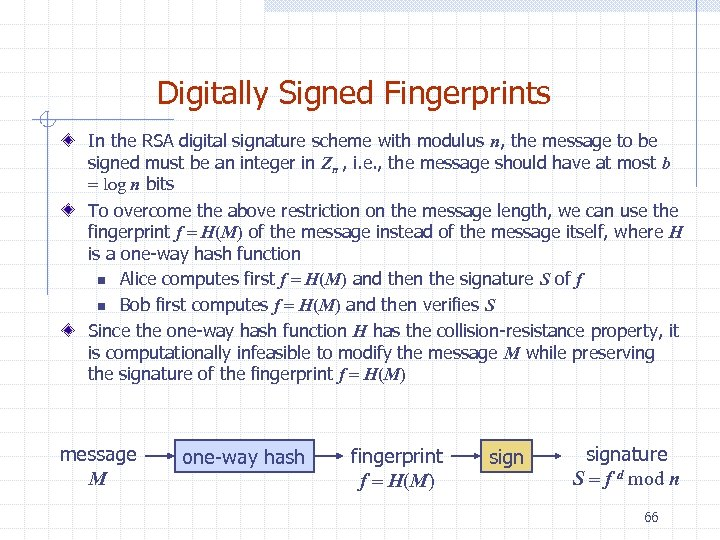Digitally Signed Fingerprints In the RSA digital signature scheme with modulus n, the message to be signed must be an integer in Zn , i. e. , the message should have at most b = log n bits To overcome the above restriction on the message length, we can use the fingerprint f = H(M) of the message instead of the message itself, where H is a one-way hash function n Alice computes first f = H(M) and then the signature S of f n Bob first computes f = H(M) and then verifies S Since the one-way hash function H has the collision-resistance property, it is computationally infeasible to modify the message M while preserving the signature of the fingerprint f = H(M) message M one-way hash fingerprint f = H(M) signature S = f d mod n 66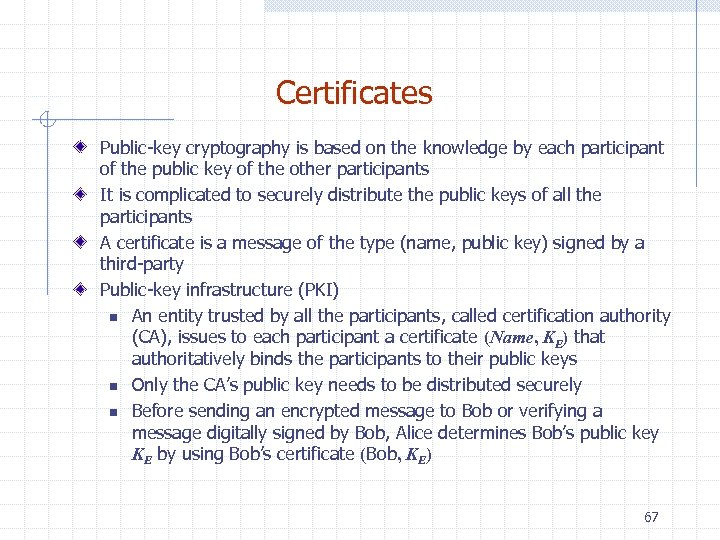Certificates Public-key cryptography is based on the knowledge by each participant of the public key of the other participants It is complicated to securely distribute the public keys of all the participants A certificate is a message of the type (name, public key) signed by a third-party Public-key infrastructure (PKI) n An entity trusted by all the participants, called certification authority (CA), issues to each participant a certificate (Name, KE) that authoritatively binds the participants to their public keys n Only the CA’s public key needs to be distributed securely n Before sending an encrypted message to Bob or verifying a message digitally signed by Bob, Alice determines Bob’s public key KE by using Bob’s certificate (Bob, KE) 67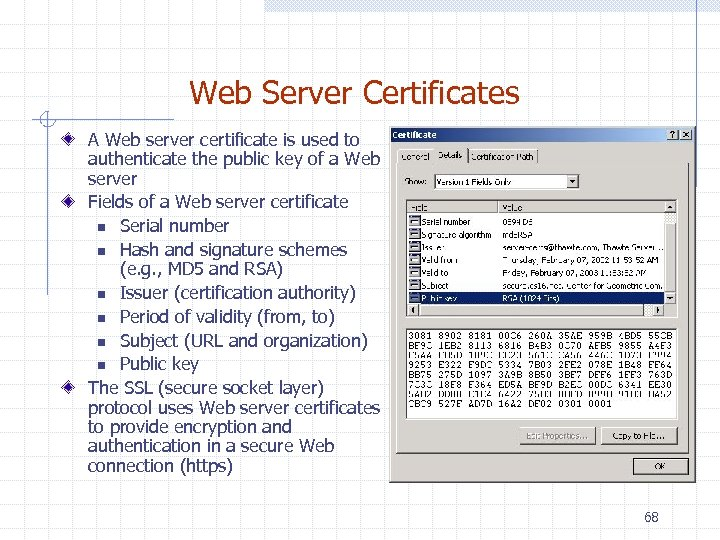Web Server Certificates A Web server certificate is used to authenticate the public key of a Web server Fields of a Web server certificate n Serial number n Hash and signature schemes (e. g. , MD 5 and RSA) n Issuer (certification authority) n Period of validity (from, to) n Subject (URL and organization) n Public key The SSL (secure socket layer) protocol uses Web server certificates to provide encryption and authentication in a secure Web connection (https) 68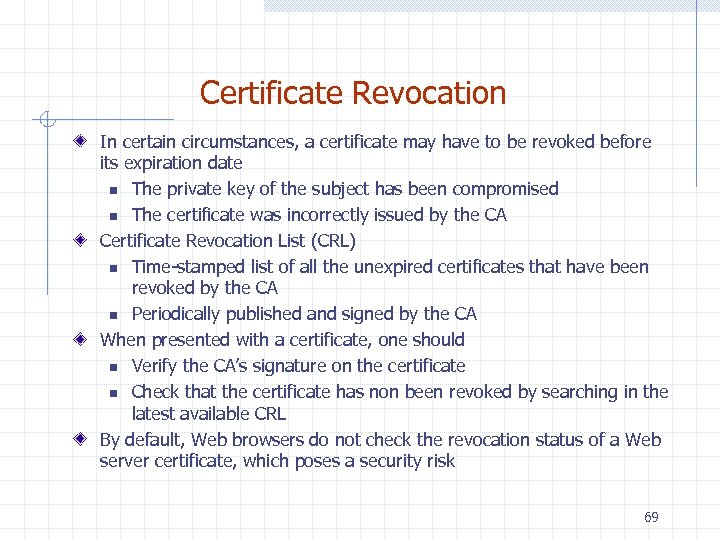Certificate Revocation In certain circumstances, a certificate may have to be revoked before its expiration date n The private key of the subject has been compromised n The certificate was incorrectly issued by the CA Certificate Revocation List (CRL) n Time-stamped list of all the unexpired certificates that have been revoked by the CA n Periodically published and signed by the CA When presented with a certificate, one should n Verify the CA’s signature on the certificate n Check that the certificate has non been revoked by searching in the latest available CRL By default, Web browsers do not check the revocation status of a Web server certificate, which poses a security risk 69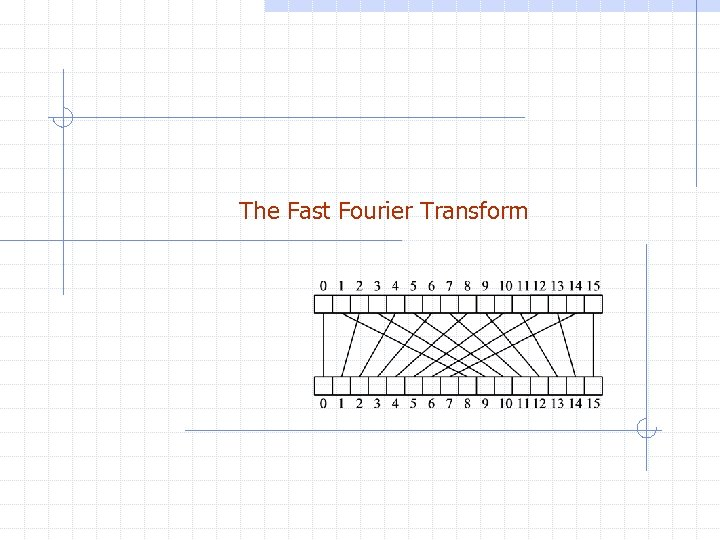The Fast Fourier Transform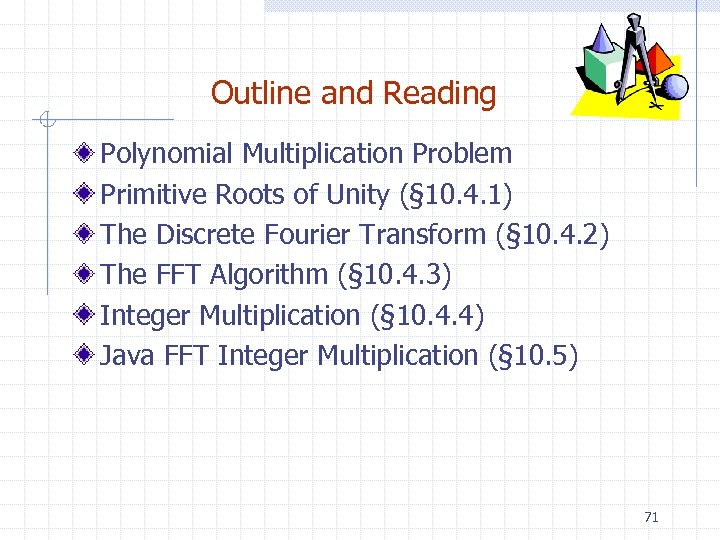Outline and Reading Polynomial Multiplication Problem Primitive Roots of Unity (§ 10. 4. 1) The Discrete Fourier Transform (§ 10. 4. 2) The FFT Algorithm (§ 10. 4. 3) Integer Multiplication (§ 10. 4. 4) Java FFT Integer Multiplication (§ 10. 5) 71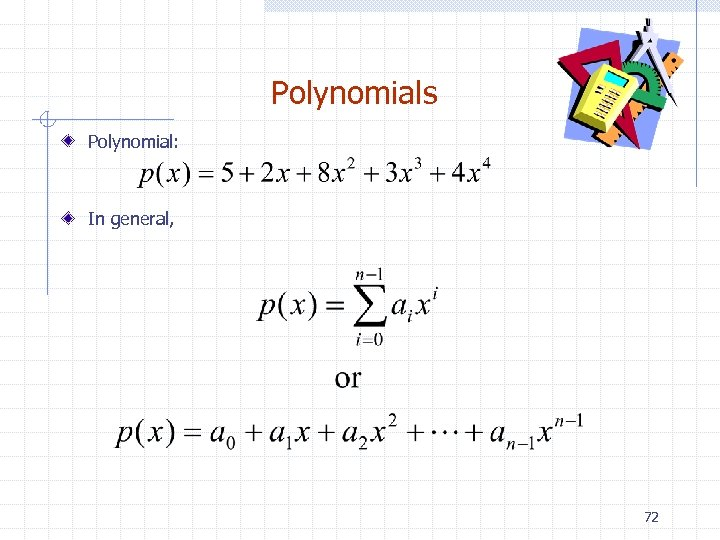Polynomials Polynomial: In general, 72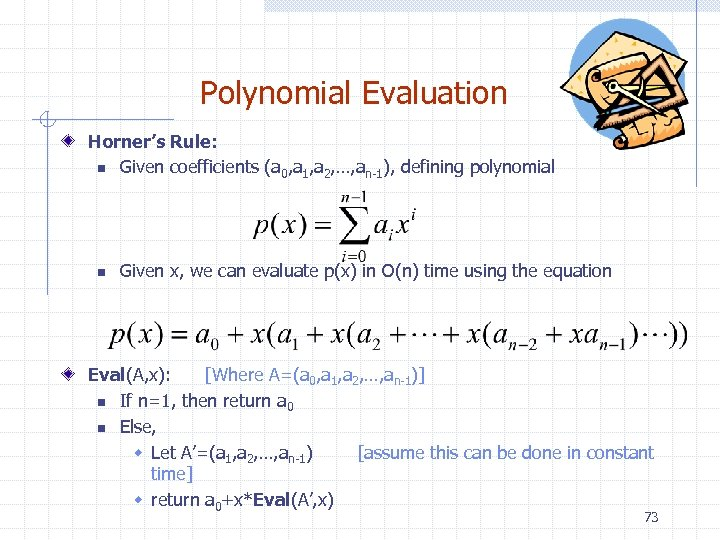Polynomial Evaluation Horner’s Rule: n Given coefficients (a 0, a 1, a 2, …, an-1), defining polynomial n Given x, we can evaluate p(x) in O(n) time using the equation Eval(A, x): [Where A=(a 0, a 1, a 2, …, an-1)] n If n=1, then return a 0 n Else, w Let A’=(a 1, a 2, …, an-1) [assume this can be done in constant time] w return a 0+x*Eval(A’, x) 73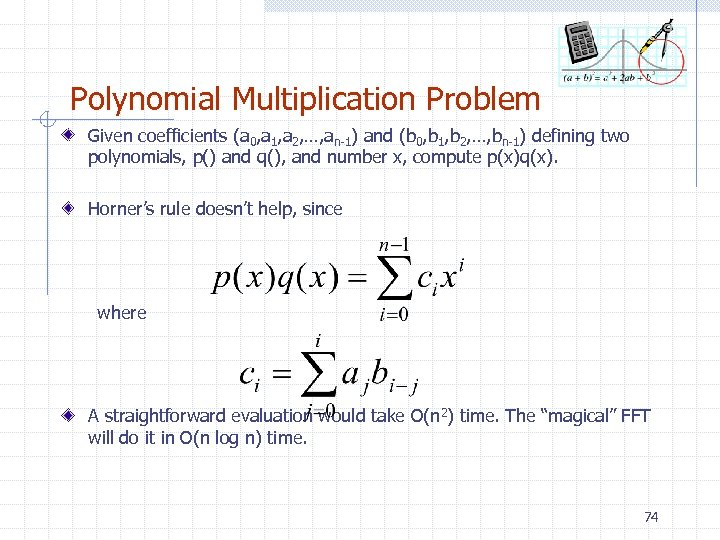Polynomial Multiplication Problem Given coefficients (a 0, a 1, a 2, …, an-1) and (b 0, b 1, b 2, …, bn-1) defining two polynomials, p() and q(), and number x, compute p(x)q(x). Horner’s rule doesn’t help, since where A straightforward evaluation would take O(n 2) time. The “magical” FFT will do it in O(n log n) time. 74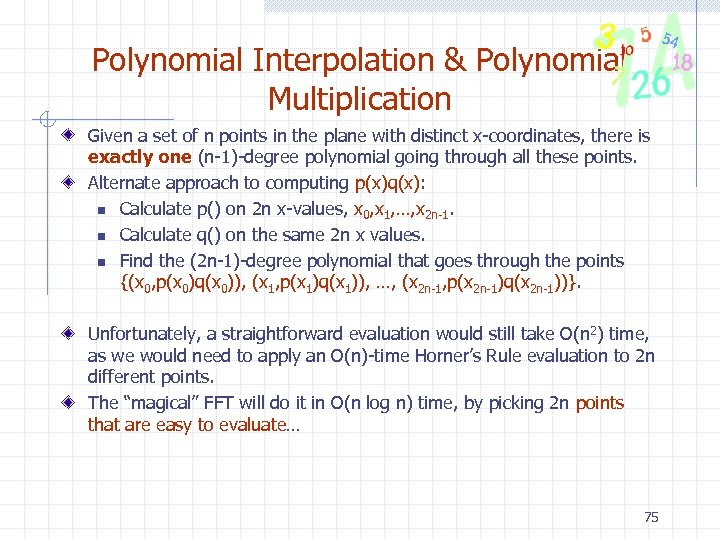Polynomial Interpolation & Polynomial Multiplication Given a set of n points in the plane with distinct x-coordinates, there is exactly one (n-1)-degree polynomial going through all these points. Alternate approach to computing p(x)q(x): n Calculate p() on 2 n x-values, x 0, x 1, …, x 2 n-1. n Calculate q() on the same 2 n x values. n Find the (2 n-1)-degree polynomial that goes through the points {(x 0, p(x 0)q(x 0)), (x 1, p(x 1)q(x 1)), …, (x 2 n-1, p(x 2 n-1)q(x 2 n-1))}. Unfortunately, a straightforward evaluation would still take O(n 2) time, as we would need to apply an O(n)-time Horner’s Rule evaluation to 2 n different points. The “magical” FFT will do it in O(n log n) time, by picking 2 n points that are easy to evaluate… 75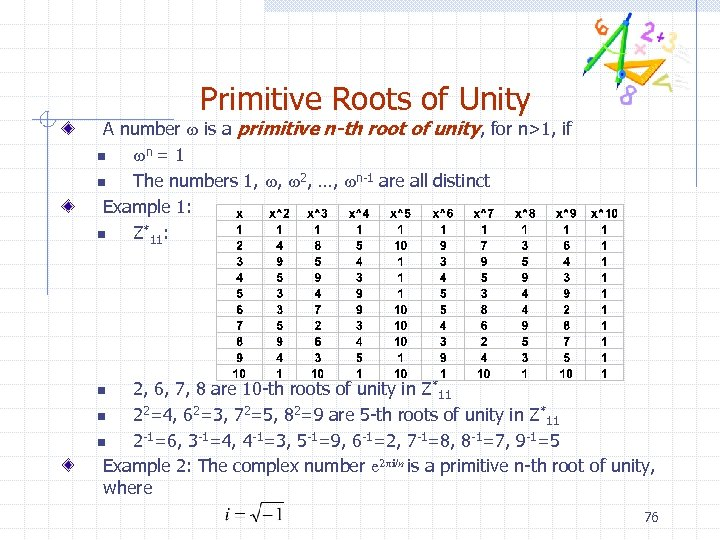Primitive Roots of Unity A number w is a primitive n-th root of unity, for n>1, if n wn = 1 n The numbers 1, w, w 2, …, wn-1 are all distinct Example 1: n Z*11: 2, 6, 7, 8 are 10 -th roots of unity in Z*11 n 22=4, 62=3, 72=5, 82=9 are 5 -th roots of unity in Z*11 n 2 -1=6, 3 -1=4, 4 -1=3, 5 -1=9, 6 -1=2, 7 -1=8, 8 -1=7, 9 -1=5 Example 2: The complex number e 2 pi/n is a primitive n-th root of unity, where n 76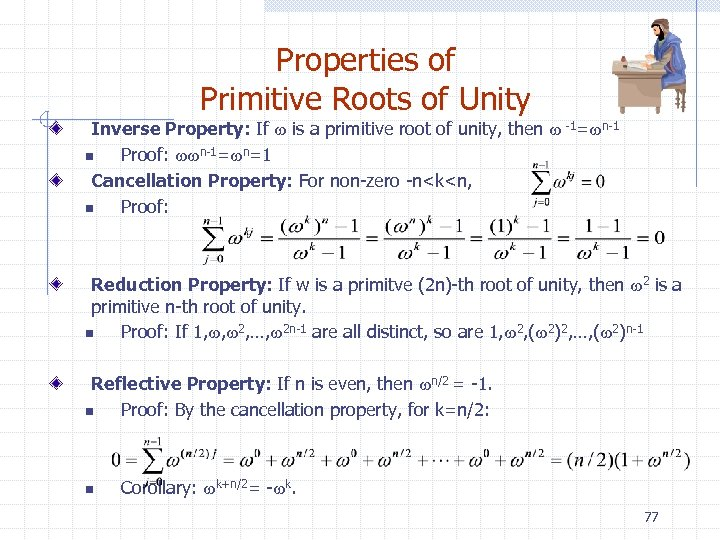Properties of Primitive Roots of Unity Inverse Property: If w is a primitive root of unity, then w -1=wn-1 n Proof: wwn-1=wn=1 Cancellation Property: For non-zero -n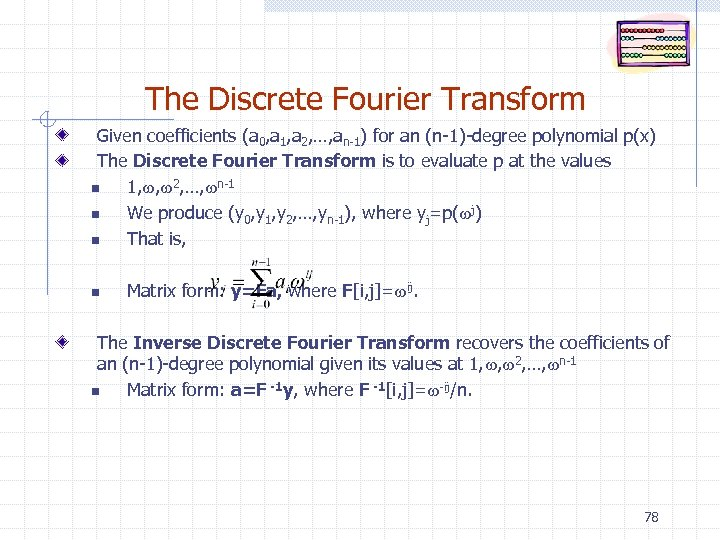The Discrete Fourier Transform Given coefficients (a 0, a 1, a 2, …, an-1) for an (n-1)-degree polynomial p(x) The Discrete Fourier Transform is to evaluate p at the values n 1, w, w 2, …, wn-1 n We produce (y 0, y 1, y 2, …, yn-1), where yj=p(wj) n That is, n Matrix form: y=Fa, where F[i, j]=wij. The Inverse Discrete Fourier Transform recovers the coefficients of an (n-1)-degree polynomial given its values at 1, w, w 2, …, wn-1 n Matrix form: a=F -1 y, where F -1[i, j]=w-ij/n. 78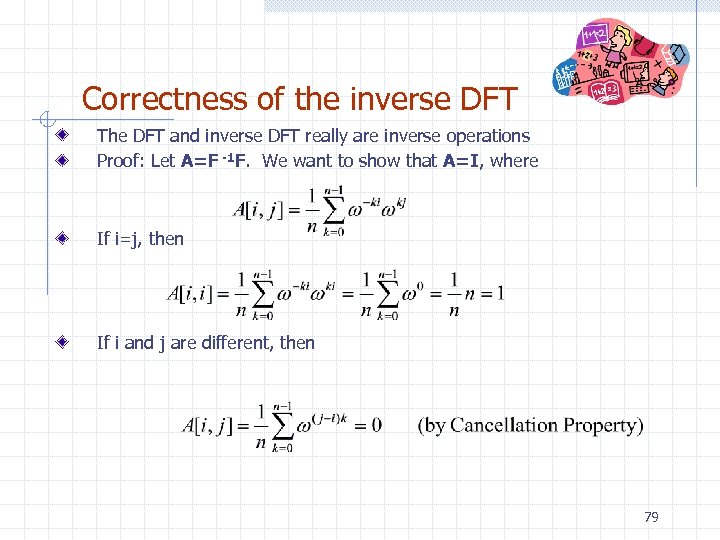Correctness of the inverse DFT The DFT and inverse DFT really are inverse operations Proof: Let A=F -1 F. We want to show that A=I, where If i=j, then If i and j are different, then 79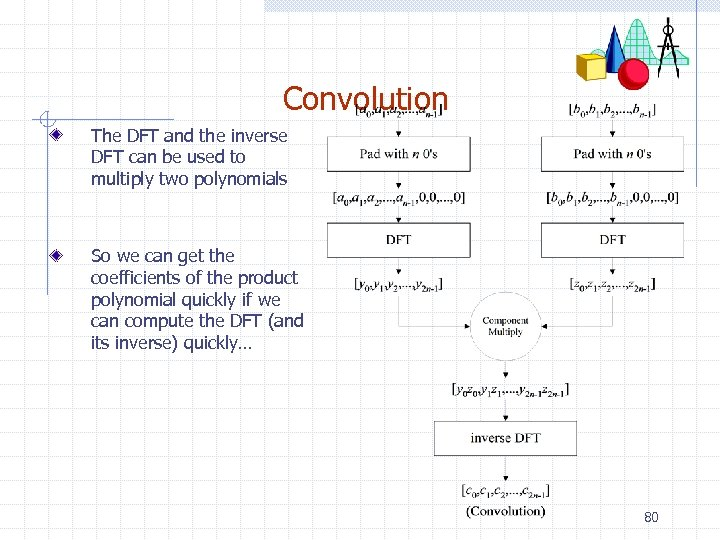Convolution The DFT and the inverse DFT can be used to multiply two polynomials So we can get the coefficients of the product polynomial quickly if we can compute the DFT (and its inverse) quickly… 80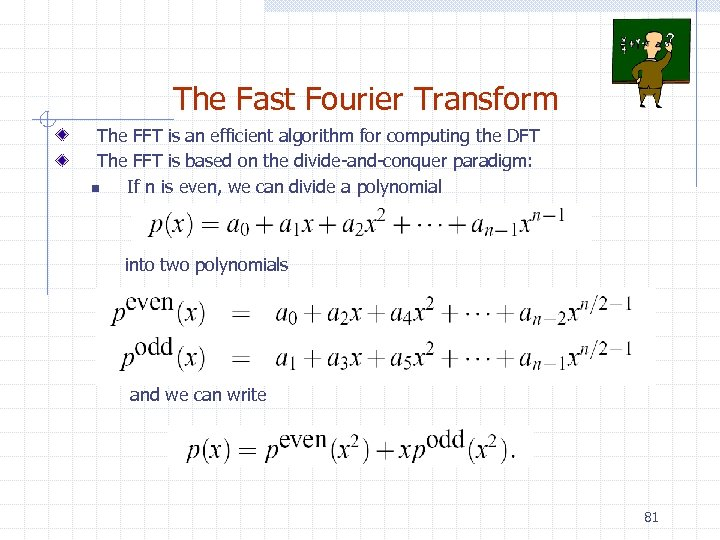The Fast Fourier Transform The FFT is an efficient algorithm for computing the DFT The FFT is based on the divide-and-conquer paradigm: n If n is even, we can divide a polynomial into two polynomials and we can write 81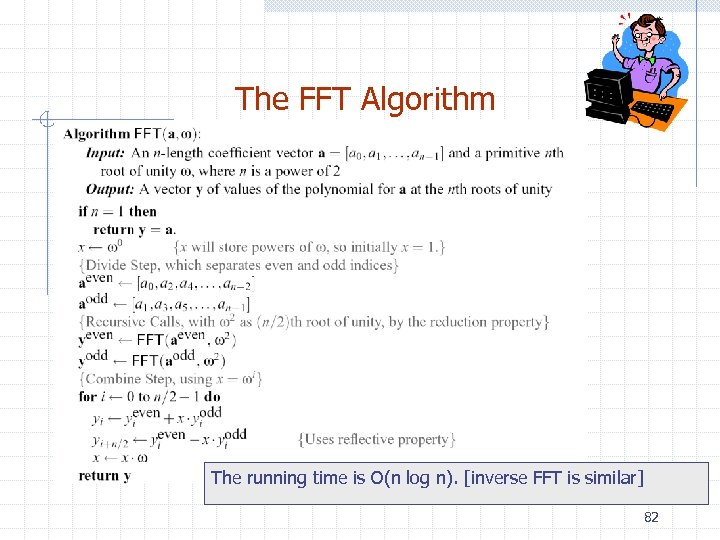The FFT Algorithm The running time is O(n log n). [inverse FFT is similar] 82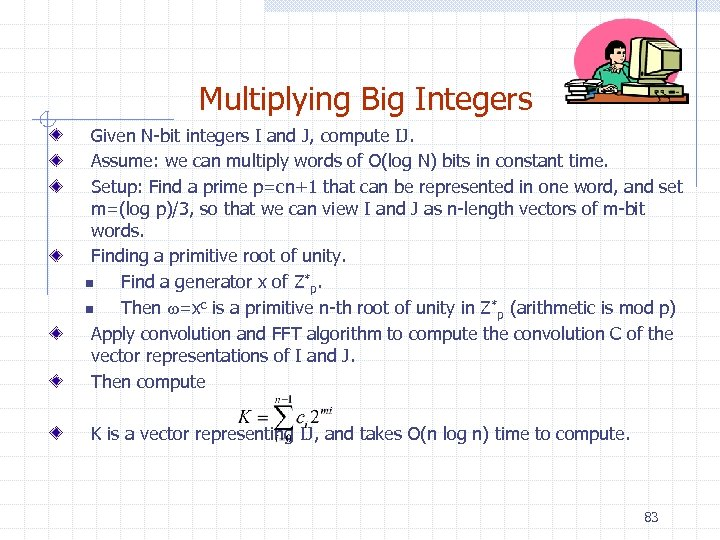Multiplying Big Integers Given N-bit integers I and J, compute IJ. Assume: we can multiply words of O(log N) bits in constant time. Setup: Find a prime p=cn+1 that can be represented in one word, and set m=(log p)/3, so that we can view I and J as n-length vectors of m-bit words. Finding a primitive root of unity. n Find a generator x of Z*p. n Then w=xc is a primitive n-th root of unity in Z*p (arithmetic is mod p) Apply convolution and FFT algorithm to compute the convolution C of the vector representations of I and J. Then compute K is a vector representing IJ, and takes O(n log n) time to compute. 83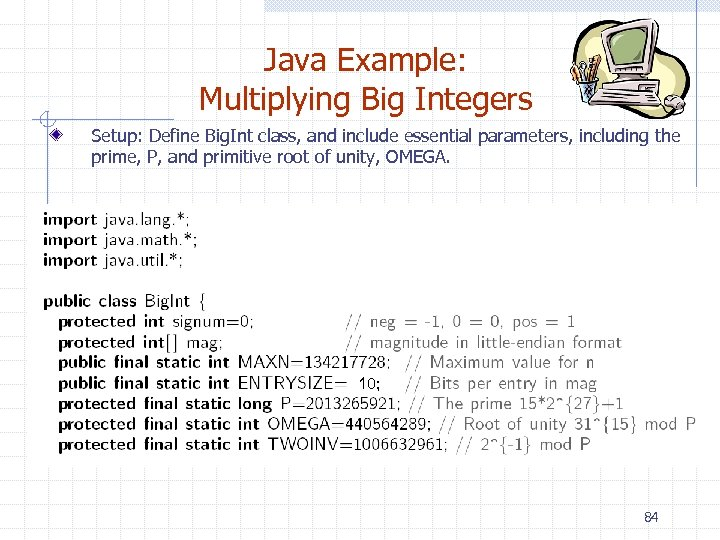Java Example: Multiplying Big Integers Setup: Define Big. Int class, and include essential parameters, including the prime, P, and primitive root of unity, OMEGA. 10; 84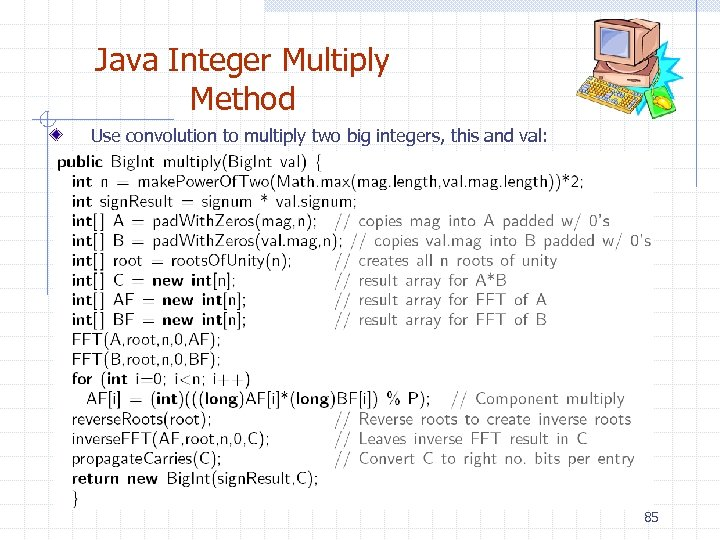Java Integer Multiply Method Use convolution to multiply two big integers, this and val: 85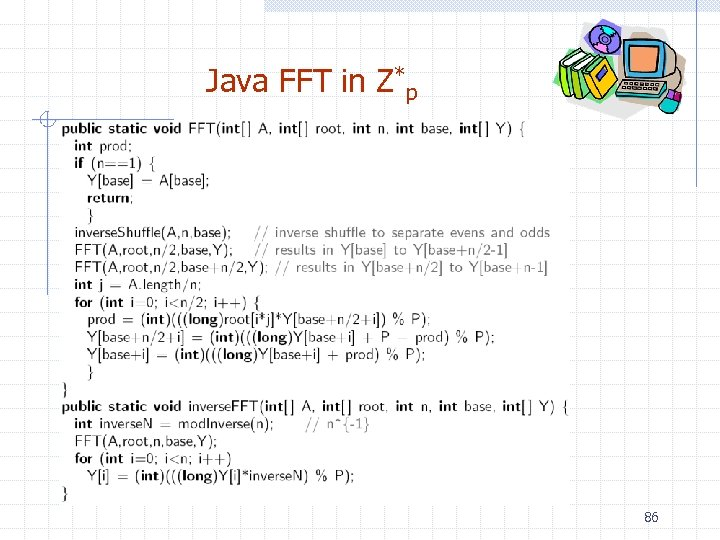Java FFT in Z*p 86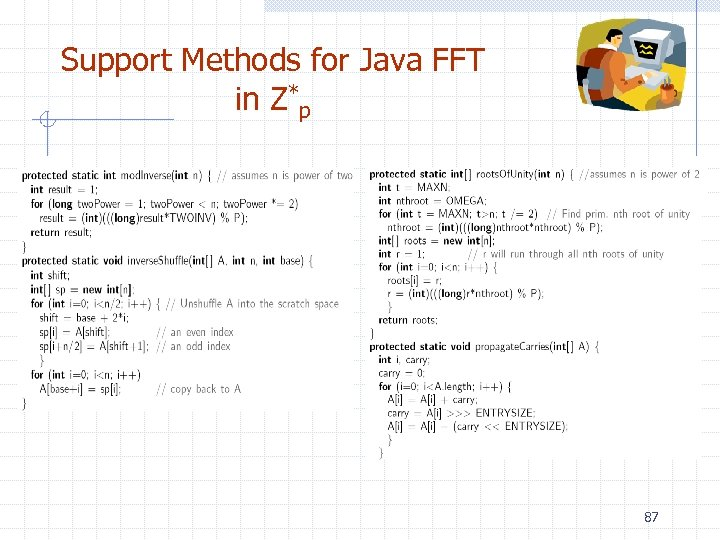Support Methods for Java FFT in Z*p 87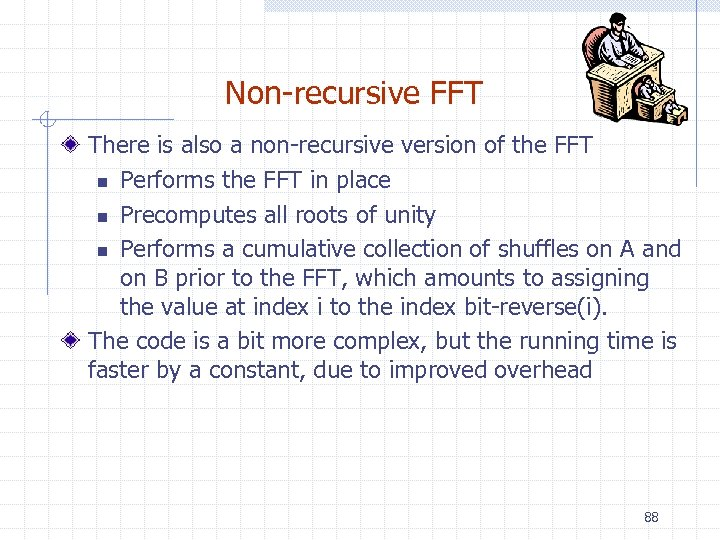Non-recursive FFT There is also a non-recursive version of the FFT n Performs the FFT in place n Precomputes all roots of unity n Performs a cumulative collection of shuffles on A and on B prior to the FFT, which amounts to assigning the value at index i to the index bit-reverse(i). The code is a bit more complex, but the running time is faster by a constant, due to improved overhead 88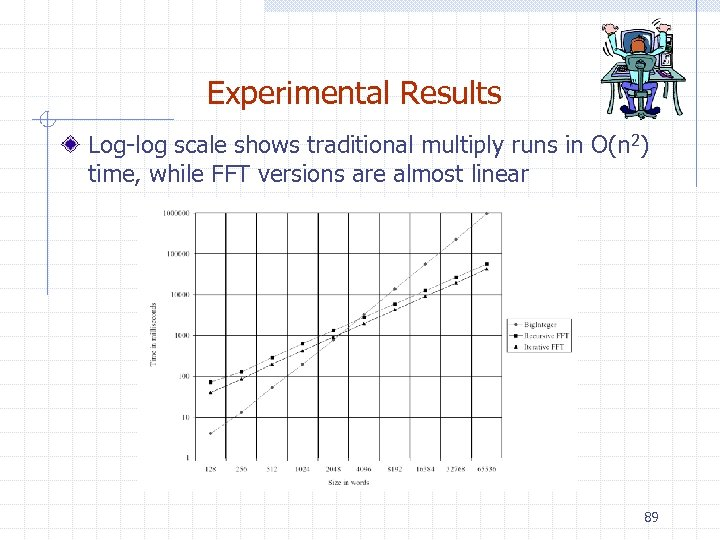Experimental Results Log-log scale shows traditional multiply runs in O(n 2) time, while FFT versions are almost linear 89